# JP4429338B2 - Motor control device, current detection unit - Google Patents

## Info

Publication number
JP4429338B2
JP4429338B2 JP2007175762A JP2007175762A JP4429338B2 JP 4429338 B2 JP4429338 B2 JP 4429338B2 JP 2007175762 A JP2007175762 A JP 2007175762A JP 2007175762 A JP2007175762 A JP 2007175762A JP 4429338 B2 JP4429338 B2 JP 4429338B2
Authority
JP
Japan
Prior art keywords
phase
current
voltage
voltage command
axis
Prior art date
Legal status (The legal status is an assumption and is not a legal conclusion. Google has not performed a legal analysis and makes no representation as to the accuracy of the status listed.)
Expired - Fee Related
Application number
JP2007175762A
Other languages
Japanese (ja)
Other versions
JP2008099542A (en
Inventor

Original Assignee

Priority date (The priority date is an assumption and is not a legal conclusion. Google has not performed a legal analysis and makes no representation as to the accuracy of the date listed.)
Filing date
Publication date
Priority to JP2006245101 priority Critical
Application filed by 三洋電機株式会社 filed Critical 三洋電機株式会社
Priority to JP2007175762A priority patent/JP4429338B2/en
Publication of JP2008099542A publication Critical patent/JP2008099542A/en
Application granted granted Critical
Publication of JP4429338B2 publication Critical patent/JP4429338B2/en
Expired - Fee Related legal-status Critical Current
Anticipated expiration legal-status Critical

• 244000171263 Ribes grossularia Species 0.000 title claims description 491
• 238000000034 methods Methods 0.000 claims description 62
• 230000000875 corresponding Effects 0.000 claims description 31
• 238000006243 chemical reactions Methods 0.000 claims description 11
• 230000001276 controlling effects Effects 0.000 claims description 5
• 239000002131 composite materials Substances 0.000 claims 1
• 238000010586 diagrams Methods 0.000 description 57
• 239000000969 carriers Substances 0.000 description 42
• 230000000051 modifying Effects 0.000 description 33
• 230000014509 gene expression Effects 0.000 description 26
• 238000005070 sampling Methods 0.000 description 23
• 238000004364 calculation methods Methods 0.000 description 22
• 238000001514 detection method Methods 0.000 description 13
• 239000003990 capacitor Substances 0.000 description 8
• 238000009499 grossing Methods 0.000 description 8
• 239000000203 mixtures Substances 0.000 description 8
• 238000004804 winding Methods 0.000 description 8
• 280000638271 Reference Point companies 0.000 description 7
• 230000001131 transforming Effects 0.000 description 7
• 230000000694 effects Effects 0.000 description 6
• 230000001264 neutralization Effects 0.000 description 5
• 230000004048 modification Effects 0.000 description 4
• 238000006011 modification reactions Methods 0.000 description 4
• 230000001809 detectable Effects 0.000 description 3
• 238000004458 analytical methods Methods 0.000 description 2
• 230000004907 flux Effects 0.000 description 2
• 230000000737 periodic Effects 0.000 description 2
• 230000001360 synchronised Effects 0.000 description 2
• 280000115721 A Line companies 0.000 description 1
• 230000037010 Beta Effects 0.000 description 1
• 239000003570 air Substances 0.000 description 1
• 230000003247 decreasing Effects 0.000 description 1
• 238000005516 engineering processes Methods 0.000 description 1
• 239000000446 fuels Substances 0.000 description 1
• 238000009413 insulation Methods 0.000 description 1
• 230000002250 progressing Effects 0.000 description 1
• 230000001052 transient Effects 0.000 description 1

## Images

•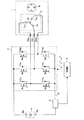•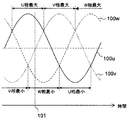•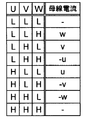•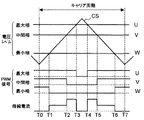•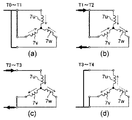•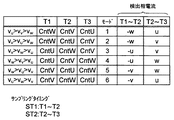•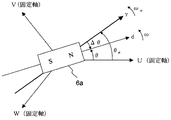•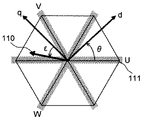•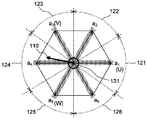•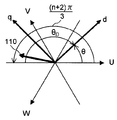•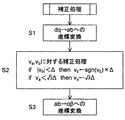•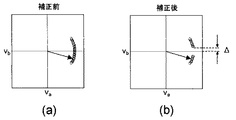•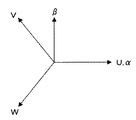•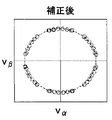•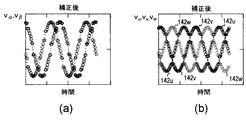•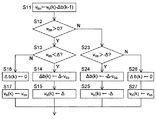•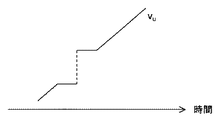•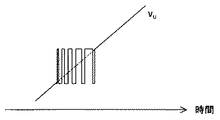•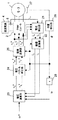•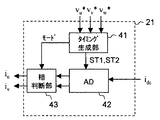•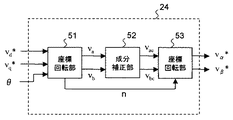•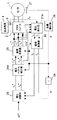•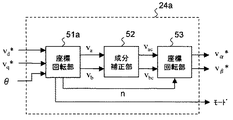•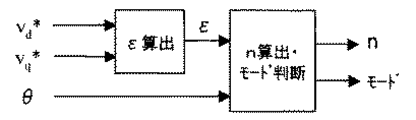•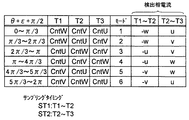•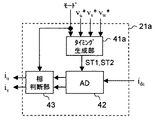•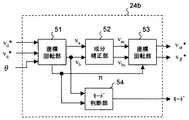•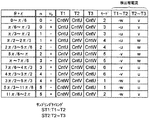•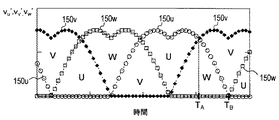•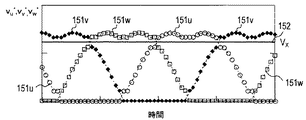•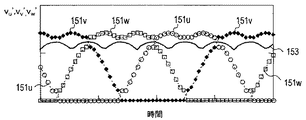•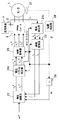•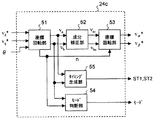•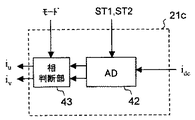•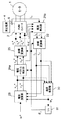•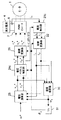•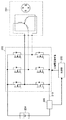•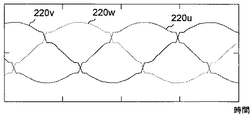•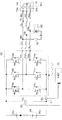•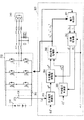•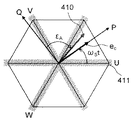••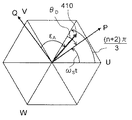••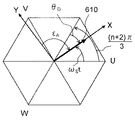•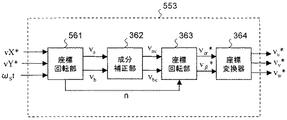## Classifications

• HELECTRICITY
• H02GENERATION; CONVERSION OR DISTRIBUTION OF ELECTRIC POWER
• H02PCONTROL OR REGULATION OF ELECTRIC MOTORS, ELECTRIC GENERATORS OR DYNAMO-ELECTRIC CONVERTERS; CONTROLLING TRANSFORMERS, REACTORS OR CHOKE COILS
• H02P21/00Arrangements or methods for the control of electric machines by vector control, e.g. by control of field orientation
• H02P21/06Rotor flux based control involving the use of rotor position or rotor speed sensors
• HELECTRICITY
• H02GENERATION; CONVERSION OR DISTRIBUTION OF ELECTRIC POWER
• H02MAPPARATUS FOR CONVERSION BETWEEN AC AND AC, BETWEEN AC AND DC, OR BETWEEN DC AND DC, AND FOR USE WITH MAINS OR SIMILAR POWER SUPPLY SYSTEMS; CONVERSION OF DC OR AC INPUT POWER INTO SURGE OUTPUT POWER; CONTROL OR REGULATION THEREOF
• H02M7/00Conversion of ac power input into dc power output; Conversion of dc power input into ac power output
• H02M7/02Conversion of ac power input into dc power output without possibility of reversal
• H02M7/04Conversion of ac power input into dc power output without possibility of reversal by static converters
• H02M7/12Conversion of ac power input into dc power output without possibility of reversal by static converters using discharge tubes with control electrode or semiconductor devices with control electrode
• H02M7/21Conversion of ac power input into dc power output without possibility of reversal by static converters using discharge tubes with control electrode or semiconductor devices with control electrode using devices of a triode or transistor type requiring continuous application of a control signal
• H02M7/217Conversion of ac power input into dc power output without possibility of reversal by static converters using discharge tubes with control electrode or semiconductor devices with control electrode using devices of a triode or transistor type requiring continuous application of a control signal using semiconductor devices only

## Description

The present invention relates to a motor control device that drives and controls a motor, and more particularly to a motor control device that employs a single shunt current detection method. The present invention also relates to a current detection unit, an inverter control device, and a grid interconnection device.

In order to control the motor by supplying three-phase AC power to the motor, the current of two phases (for example, U-phase current and V-phase current) is detected among the three phases U-phase, V-phase and W-phase. There is a need to. Usually, two current sensors (such as a current transformer) are used to detect the current for two phases. However, the use of the two current sensors increases the cost of the entire system incorporating the motor.

For this reason, conventionally, a method has been proposed in which a bus current (DC current) between an inverter and a DC power supply is detected by a single current sensor, and currents for two phases are detected from the detected bus current. This method is also called a single shunt current detection method (single shunt current detection method), and the basic principle of this method is described in, for example, Patent Document 1 below.

FIG. 37 shows an overall block diagram of a conventional motor drive system that employs a single shunt current detection method. The inverter (PWM inverter) 202 includes a half-bridge circuit having an upper arm and a lower arm for three phases, and switches each arm in accordance with a three-phase voltage command value given from the control unit 203, thereby Is converted into a three-phase AC voltage. The three-phase AC voltage is supplied to a three-phase permanent magnet synchronous motor 201, and the motor 201 is driven and controlled.

A line connecting each lower arm in the inverter 202 and the DC power supply 204 is referred to as a bus 213. The current sensor 205 transmits a signal representing the bus current flowing through the bus 213 to the control unit 203. The control unit 203 samples the output signal of the current sensor 205 at an appropriate timing, so that the phase current of the phase with the maximum voltage level (maximum phase) and the phase current of the phase with the minimum (minimum phase), that is, The current for two phases is detected.

When the voltage level of each phase is sufficiently far from each other, the current for two phases can be detected by the above processing, but when the maximum phase and the intermediate phase of the voltage approach each other, or the minimum phase and the intermediate phase of the voltage When the phases approach, current for two phases cannot be detected. The description of the one-shunt current detection method including the description that the current for two phases cannot be detected will be given later with reference to FIGS.

In view of this, in Patent Document 2 below, in a one-shunt current detection method, a three-phase current is estimated from past current information during a period in which a current for two phases cannot be detected. More specifically, the three-phase current is estimated by inversely converting the d-axis q-axis current obtained by converting the past three-phase current into three phases.

When estimation is based only on the past three-phase current, a certain degree of estimation accuracy can be expected in the steady state, but in the transient state, the effect of the voltage applied to the motor is not reflected in the estimation, so good estimation can be expected. Absent. Even if the current information for the remaining phase is estimated using the current information for one phase that can be detected, the current information for the one phase cannot reflect the effect of the applied voltage, and it is difficult to make a good estimation.

In addition, when the current estimation method as described in Patent Document 2 is adopted, since estimation is performed instead of detection, an error is included in the current value used for vector control. This error is not desirable for smooth driving of the motor.

Therefore, in the one-shunt current detection method, a method of correcting the pulse width of the PWM signal for each arm in the inverter based on the three-phase gate signal during a period in which current for two phases cannot be detected is proposed. (For example, refer to Patent Document 3 below).

A correction example of a general voltage command value (pulse width) corresponding to this correction is shown in FIG. In FIG. 38, the horizontal axis represents time, and 220u, 220v, and 220w represent voltage levels of the U phase, the V phase, and the W phase. Since the voltage level of each phase follows the voltage command value (pulse width) for each phase, both can be considered equivalent. As shown in FIG. 38, the voltage command value (pulse width) of each phase is corrected so that the “maximum phase and intermediate phase” and “minimum phase and intermediate phase” of the voltage do not approach a predetermined interval or less. As a result, the voltages of the phases do not approach so much that the current for two phases cannot be detected, and the current for two phases can be detected stably.

However, in the method described in Patent Document 3, it is necessary to determine the correction amount from the relationship between the voltage command values (pulse widths) for the three phases. In particular, when the applied voltage is low, correction is required for all three phases. As a result, the correction process becomes complicated.

Note that Patent Document 1 below does not describe a countermeasure method for the case where the current for two phases cannot be detected. Non-Patent Document 1 below discloses a control technique for a three-phase inverter for grid connection.

Japanese Patent No. 2712470 JP 2004-64903 A JP 2003-189670 A Yamada, et al., "Current Controlled Type Sinusoidal Voltage Interconnecting Three-Phase Inverter", Proceedings of National Conference of the IEEJ, IEEJ, 2007 3 Month, 4th volume, 4-076, p. 115

As described above, when the one-shunt current detection method is adopted, the correction as shown in FIG. 38 is effective, but at the same time, a desired correction for maintaining the motor current detectable state by a simpler process. The technology to achieve this is eagerly desired. Moreover, although the prior art regarding motor control was demonstrated, the problem similar to the problem which the prior art has may arise with respect to a grid connection system etc.

Accordingly, the present invention provides a motor control device and a motor drive system capable of realizing a desired correction for maintaining a motor current detectable state by a simple process when a single shunt current detection method is employed. The purpose is to do. In addition, the present invention adopts a current detection method corresponding to the one shunt current detection method, and realizes a desired correction for maintaining a detectable state of the inverter output current, an inverter control device, and a system connection. An object is to provide a system device.

In order to achieve the above object, a motor control device according to the present invention includes current detection means for detecting, as a detection current, a current flowing between an inverter that drives a three-phase motor and a DC power source, In a motor control device that detects a motor current flowing through the motor from a detected current and controls the motor via the inverter based on the motor current, an applied voltage to the motor should follow the motor current A voltage command vector creating means for creating a voltage command vector representing a voltage vector; and a voltage command vector correcting means for correcting the created voltage command vector. The motor is controlled according to the corrected voltage command vector. It is characterized by doing.

For example, the voltage command vector is a voltage command vector on a rotation coordinate, and the voltage command vector correction means converts the voltage command vector on the rotation coordinate into a three-phase voltage command value on a three-phase fixed coordinate. In the process, the voltage command vector on the rotation coordinate is corrected, and the motor control device controls the motor by supplying the inverter with the three-phase voltage command corresponding to the corrected voltage command vector. .

More specifically, for example, the voltage command vector is a two-phase voltage command on the ab coordinate that rotates stepwise every electrical angle 60 degrees in accordance with the phase of the voltage command vector with a predetermined fixed axis as a reference. Is a vector.

Thereby, a desired correction can be realized by a simple process of correcting the coordinate axis component of the voltage command vector.

That is, for example, the voltage command vector correction means determines whether or not correction is necessary based on the size of the coordinate axis component that forms the two-phase voltage command vector on the ab coordinate. The voltage command vector is corrected by correcting.

Further, for example, the motor control device determines the phase of the current flowing as the detection current based on the generated voltage command vector, and detects the motor current according to the determination result.

More specifically, the following method can be considered as a method of the above determination based on the voltage command vector. For example, the phase of the current flowing as the detection current is determined based on the phase of the voltage command vector with a predetermined fixed axis as a reference.

Instead of this, for example, the phase of the current flowing as the detection current based on the coordinate axis component of the voltage command vector in the ab coordinate and a variable according to the phase difference between the coordinate axis of the ab coordinate and a predetermined fixed axis Judging.

And, for example, the motor control device further includes a three-phase voltage command value creating means for creating a three-phase voltage command value from the corrected voltage command vector, and based on the determination result and the three-phase voltage command value, The timing for detecting the detected current is determined, the motor current is detected from the detected current detected at that timing, and the motor is controlled by supplying the three-phase voltage command value to the inverter.

Further, for example, a timing for detecting the detected current may be determined based on the magnitude of the generated voltage command vector, and the motor current may be detected from the detected current detected at that timing.

Further, for example, a timing for detecting the detection current may be determined based on a coordinate axis component of the voltage command vector in the ab coordinate, and the motor current may be detected from the detection current detected at the timing. .

In order to achieve the above object, a motor drive system according to the present invention includes a three-phase motor, an inverter that drives the motor, and any of the above that controls the motor by controlling the inverter. And a motor control device as described above.

In order to achieve the above object, a current detection unit according to the present invention includes current detection means for detecting a current flowing between a three-phase inverter and a DC power supply as a detection current, and the detected current detected In the current detection unit for detecting the three-phase current of the inverter from the voltage command vector creating means for creating a voltage command vector representing the vector of the voltage that the three-phase voltage of the inverter should follow, and the created voltage command vector Voltage command vector correction means for correcting the voltage, and the inverter is controlled in accordance with the corrected voltage command vector.

For example, in the current detection unit, the voltage command vector is a voltage command vector on a rotation coordinate, and the voltage command vector correction means converts the voltage command vector on the rotation coordinate to a three-phase fixed coordinate on three phases. In the process of converting to a voltage command value, the voltage command vector on the rotation coordinate is corrected, and the inverter is supplied with the three-phase voltage command value corresponding to the corrected voltage command vector. Be controlled.

More specifically, for example, in the current detection unit, the voltage command vector is an ab coordinate that rotates stepwise at every electrical angle of 60 degrees according to the phase of the voltage command vector with respect to a predetermined fixed axis. Is a two-phase voltage command vector.

Thereby, a desired correction can be realized by a simple process of correcting the coordinate axis component of the voltage command vector.

That is, for example, in the current detection unit, the voltage command vector correction means determines whether or not correction is necessary based on the size of the coordinate axis component forming the two-phase voltage command vector on the ab coordinate, and correction is necessary. In this case, the voltage command vector is corrected by correcting the coordinate axis component.

An inverter control device according to the present invention is an inverter control device that includes the above-described current detection unit and controls the inverter based on the detected three-phase current, wherein the voltage command vector creating means includes the three The voltage command vector is generated based on a phase current.

For example, the inverter control device further includes current conversion means for converting the three-phase current into effective current and reactive current with reference to the phase of the output voltage of the inverter, and the voltage command vector creation means includes the effective current The voltage command vector is generated based on the reactive current.

Further, a grid interconnection device according to the present invention includes the inverter control device and the inverter described above, converts a DC voltage from the DC power source into a three-phase AC voltage by the inverter, and converts it into an external three-phase AC power system. AC power based on the AC voltage is supplied to a load while being connected.

According to the present invention, when the single shunt current detection method is adopted, desired correction can be realized by a simple process.

Hereinafter, embodiments of the present invention will be specifically described with reference to the drawings. In each of the drawings to be referred to, the same part is denoted by the same reference numeral, and redundant description regarding the same part is omitted in principle.

<< First Embodiment >>
First, a first embodiment of the present invention will be described. Later, first to fifth examples of the first embodiment will be described. First, matters common to the examples and items to be referred to in the examples will be described. FIG. 1 is a block diagram of a motor drive system according to the first embodiment.

1 includes a three-phase permanent magnet synchronous motor 1 (hereinafter simply referred to as “motor 1”), a PWM (Pulse Width Modulation) inverter 2 (hereinafter simply referred to as “inverter 2”), and a control unit. 3, a DC power supply 4, and a current sensor 5. The DC power supply 4 outputs a DC voltage between the positive output terminal 4a and the negative output terminal 4b with the negative output terminal 4b on the low voltage side. The motor drive system of FIG. 1 employs a single shunt current detection method.

The motor 1 includes a rotor 6 provided with permanent magnets, and a stator 7 provided with U-phase, V-phase, and W-phase armature windings 7u, 7v, and 7w. The armature windings 7u, 7v and 7w are Y-connected around the neutral point 14. In the armature windings 7u, 7v, and 7w, the non-connection ends on the opposite side of the neutral point 14 are connected to the terminals 12u, 12v, and 12w, respectively.

The inverter 2 includes a U-phase half-bridge circuit, a V-phase half-bridge circuit, and a W-phase half-bridge circuit. Each half-bridge circuit has a pair of switching elements. In each half bridge circuit, the pair of switching elements are connected in series between the positive output terminal 4a and the negative output terminal 4b of the DC power supply 4, and a DC voltage from the DC power supply 4 is applied to each half bridge circuit.

The U-phase half-bridge circuit includes a high-voltage side switching element 8u (hereinafter also referred to as an upper arm 8u) and a low-voltage side switching element 9u (hereinafter also referred to as a lower arm 9u). The V-phase half-bridge circuit includes a high-voltage side switching element 8v (hereinafter also referred to as an upper arm 8v) and a low-voltage side switching element 9v (hereinafter also referred to as a lower arm 9v). The W-phase half-bridge circuit includes a high-voltage side switching element 8w (hereinafter also referred to as an upper arm 8w) and a low-voltage side switching element 9w (hereinafter also referred to as a lower arm 9w). Further, the switching elements 8u, 8v, 8w, 9u, 9v and 9w are connected in parallel to the diodes 10u, 10v, 10w, 11u, respectively, with the direction from the low voltage side to the high voltage side of the DC power supply 4 as the forward direction. 11v and 11w are connected. Each diode functions as a freewheeling diode.

The connection point between the upper arm 8u and the lower arm 9u connected in series, the connection point between the upper arm 8v and the lower arm 9v connected in series, and the connection point between the upper arm 8w and the lower arm 9w connected in series are respectively the terminal 12u. , 12v and 12w. In FIG. 1, field effect transistors are shown as the switching elements, but they can be replaced with IGBTs (insulated gate bipolar transistors) or the like.

The inverter 2 generates a PWM signal (pulse width modulation signal) for each phase based on the three-phase voltage command value given from the control unit 3, and uses the PWM signal as a control terminal (base) for each switching element in the inverter 2. (Or gate), each switching element is switched. Three-phase voltage values supplied from the controller 3 to the inverter 2 is composed of a U-phase voltage command values v u *, V-phase voltage value v v * and the W-phase voltage command value v w *, v u * , V v * and v w * represent voltage levels (voltage values) of the U-phase voltage v u , the V-phase voltage v v and the W-phase voltage v w , respectively. Then, the inverter 2 controls ON (conduction) or OFF (non-conduction) of each switching element based on v u * , v v *, and v w * .

Neglecting the dead time to prevent the upper and lower arms of the same phase from turning on at the same time, in each half-bridge circuit, when the upper arm is on, the lower arm is off and the upper arm is off When, the lower arm is on. In the following description, the dead time is ignored.

The DC voltage from the DC power supply 4 applied to the inverter 2 is converted into, for example, a PWM-modulated (pulse width modulated) three-phase AC voltage by the switching operation of each switching element in the inverter 2. When the three-phase AC voltage is applied to the motor 1, a current corresponding to the three-phase AC voltage flows through each armature winding (7u, 7v, and 7w) to drive the motor 1.

Current sensor 5 detects a current flowing through bus 13 of inverter 2 (hereinafter referred to as “bus current”). Since the bus current has a direct current component, it can be interpreted as a direct current. In the inverter 2, the lower voltage sides of the lower arms 9 u, 9 v and 9 w are connected in common and connected to the negative output terminal 4 b of the DC power supply 4. A wiring to which the low voltage sides of the lower arms 9 u, 9 v and 9 w are commonly connected is a bus 13, and the current sensor 5 is interposed in series with the bus 13. The current sensor 5 transmits a signal representing the current value of the detected bus current (detected current) to the control unit 3. The controller 3 generates and outputs the three-phase voltage command value while referring to the output signal of the current sensor 5 and the like. The current sensor 5 is, for example, a shunt resistor or a current transformer. Also, not the wiring (bus 13) connecting the low voltage side of the lower arms 9u, 9v and 9w and the negative output terminal 4b, but the high voltage side of the upper arms 8u, 8v and 8w and the positive output terminal 4a. You may make it provide the current sensor 5 in the wiring to perform.

Here, the relationship between the bus current and the phase current flowing through the armature winding of each phase will be described with reference to FIGS. The currents that flow through the armature windings 7u, 7v, and 7w are referred to as U-phase current, V-phase current, and W-phase current, respectively (or they are collectively referred to) as phase currents (see FIG. 1). ). In the phase current, the polarity of the current flowing in the direction from the terminal 12u, 12v or 12w to the neutral point 14 is positive, and the polarity of the current flowing in the direction flowing out from the neutral point 14 is negative.

FIG. 2 shows a typical example of a three-phase AC voltage applied to the motor 1. In FIG. 2, 100u, 100v, and 100w represent waveforms of the U-phase voltage, the V-phase voltage, and the W-phase voltage that are to be applied to the motor 1, respectively. Each of the U-phase voltage, V-phase voltage, and W-phase voltage (or collectively) is referred to as a phase voltage. When a sinusoidal current is supplied to the motor 1, the output voltage of the inverter 2 is sinusoidal. The phase voltages in FIG. 2 are ideal sine waves, but in the present embodiment, distortion is actually applied to the sine waves (details will be described later).

As shown in FIG. 2, the level relationship of the voltage level among the U-phase voltage, the V-phase voltage, and the W-phase voltage changes with time. This level relationship is determined by the three-phase voltage command value, and the inverter 2 determines the energization pattern for each phase according to the three-phase voltage command value. FIG. 3 shows this energization pattern as a table. The energization patterns are represented in the first column to the third column from the left side of FIG. The fourth column will be described later.

In the energization pattern,
The energization pattern “LLL” in which the lower arms of the U, V, and W phases are all on,
An energization pattern “LLH” in which the upper arm of the W phase is on and the lower arm of the U and V phases is on,
An energization pattern “LHL” in which the upper arm of the V phase is on and the lower arm of the U and W phases is on,
An energization pattern “LHH” in which the upper arm of the V and W phases is on and the lower arm of the U phase is on,
An energization pattern “HLL” in which the upper arm of the U phase is on and the lower arm of the V and W phases is on,
An energization pattern “HLH” in which the upper arm of the U and W phases is on and the lower arm of the V phase is on,
An energization pattern “HHL” in which the upper arm of the U and V phases is on and the lower arm of the W phase is on,
The energization pattern “HHH” in which the upper arms of the U, V, and W phases are all on,
(The upper arm and lower arm symbols (8u, etc.) are omitted).

FIG. 4 shows the relationship between the voltage level of each phase voltage and the carrier signal in the case of performing three-phase modulation, and the waveforms of the PWM signal and bus current corresponding to the relationship. Although the level relationship of the voltage level of each phase voltage varies in various ways, FIG. 4 focuses on a certain timing 101 shown in FIG. That is, FIG. 4 shows a case where the voltage level of the U-phase voltage is maximum and the voltage level of the W-phase voltage is minimum. The phase with the maximum voltage level is called “maximum phase”, the phase with the minimum voltage level is called “minimum phase”, and the phase with the voltage level not maximum or minimum is called “intermediate phase”. In the state shown in FIG. 4, the maximum phase, the intermediate phase, and the minimum phase are the U phase, the V phase, and the W phase, respectively. In FIG. 4, symbol CS represents a carrier signal to be compared with the voltage level of each phase voltage. The carrier signal is a periodic triangular wave signal, and the period of the signal is called a carrier period. Since the carrier period is much shorter than the period of the three-phase AC voltage shown in FIG. 2, if the triangular wave of the carrier signal shown in FIG. 4 is represented on FIG. 2, the triangular wave becomes a single line. appear.

The relationship between the phase current and the bus current will be described with further reference to FIGS. FIG. 5 is an equivalent circuit around the armature winding at each timing of FIG.

The start timing of each carrier period, that is, the timing at which the carrier signal is at the lowest level is called T0. At the timing T0, the upper arms (8u, 8v and 8w) of the respective phases are turned on. In this case, as shown in FIG. 5 (a), a short circuit is formed and no current flows in or out of the DC power supply 4, so that the bus current is zero.

The inverter 2 compares the voltage level of each phase voltage with the carrier signal with reference to v u * , v v *, and v w * . Then, in the process of increasing the level (voltage level) of the carrier signal, when the voltage level of the minimum phase reaches the timing T1 when it intersects with the carrier signal, the lower arm of the minimum phase is turned on, as shown in FIG. The minimum phase current flows as the bus current. In the example shown in FIG. 4, since the lower arm 9w of the W phase is turned on from the timing T1 to the timing T2 described later, the W phase current (polarity is negative) flows as the bus current.

Further, when the level of the carrier signal rises and reaches a timing T2 at which the voltage level of the intermediate phase intersects with the carrier signal, the upper arm of the maximum phase is turned on and the lower arm of the intermediate phase and the minimum phase are turned on. As shown in (c), the maximum phase current flows as the bus current. In the example shown in FIG. 4, the U-phase upper arm 8u is on and the V-phase and W-phase lower arms 9v and 9w are on during the period from timing T2 to timing T3 described later. Current (polarity is positive) flows as bus current.

When the carrier signal level further rises and the timing T3 at which the maximum phase voltage level intersects with the carrier signal is reached, the lower arms of all phases are turned on, and as shown in FIG. Since it is formed and no current flows into and out of the DC power supply 4, the bus current is zero.

After the carrier signal reaches the maximum level at an intermediate timing between timing T3 and timing T4 described later, the level of the carrier signal decreases. In the process of decreasing the level of the carrier signal, the states shown in FIGS. 5D, 5C, 5B, and 5A are visited in this order. That is, in the lowering process of the carrier signal level, the timing when the maximum phase voltage level intersects the carrier signal is T4, the timing when the intermediate phase voltage level intersects the carrier signal is T5, and the minimum phase voltage level is the carrier signal. Assuming that the intersection timing is T6 and the next carrier cycle start timing is T7, the timing T4-T5, the timing T5-T6, and the timing T6-T7 are between the timing T2-T3 and the timing T1-T2, respectively. The same energization pattern as that between the timings T0 and T1 is obtained.

Therefore, for example, if the bus current is detected between timings T1 and T2 or between T5 and T6, the minimum phase current can be detected from the bus current, and the bus current is detected between timings T2 and T3 or between T4 and T5. Then, the maximum phase current can be detected from the bus current. The intermediate phase current can be obtained by calculation using the fact that the sum of the three phase currents becomes zero. In the fourth column of the table of FIG. 3, the phase of the current that flows as the bus current in each energization pattern is shown with a current polarity. For example, in the energization pattern “HHL” corresponding to the eighth row in the table of FIG. 3, a W-phase current (negative polarity) flows as the bus current.

The period excluding the period between the timings T1 and T6 from the carrier period represents the pulse width of the PWM signal for the minimum phase, and the period excluding the period between the timings T2 and T5 from the carrier period is for the intermediate phase. This represents the pulse width of the PWM signal, and the period excluding the period between timings T3 and T4 from the carrier period represents the pulse width of the PWM signal for the maximum phase.

The case where the U phase is the maximum phase and the W phase is the minimum phase has been described as an example, but there are six combinations of the maximum phase, the intermediate phase, and the minimum phase. FIG. 6 shows this combination as a table. When the U-phase voltage, V-phase voltage, and W-phase voltage are represented by v u , v v, and v w , respectively,
The state in which v u > v v > v w is satisfied is the first mode,
The state in which v v > v u > v w is established is the second mode,
The state in which v v > v w > v u is established is the third mode,
The state in which v w > v v > v u is established is the fourth mode,
The state in which v w > v u > v v is established is the fifth mode,
The state in which v u > v w > v v is established is the sixth mode,
Call it. The examples shown in FIGS. 4 and 5 correspond to the first mode. FIG. 6 also shows the phase of current detected in each mode.

Specifically, the U-phase voltage command value v u * , the V-phase voltage command value v v *, and the W-phase voltage command value v w * are expressed as counter setting values CntU, CntV, and CntW, respectively. The higher the phase voltage, the greater the set value. For example, in the first mode, CntU>CntV> CntW is satisfied.

A counter (not shown) provided in the control unit 3 counts up the count value from 0 with respect to the timing T0 for each carrier period. Then, when the count value reaches CntW, the W-phase upper arm 8w is switched from the on state to the lower arm 9w, and when the count value reaches CntV, the V-phase upper arm 8v. The lower arm 9v is switched from the ON state to the ON state, and when the count value reaches CntU, the U-phase upper arm 8u is switched from the ON state to the lower arm 9u. After the carrier signal reaches the maximum level, the count value is counted down and the reverse switching operation is performed.

Therefore, in the first mode, the time when the counter value reaches CntW corresponds to timing T1, the time when CntV is reached corresponds to timing T2, and the time when CntU reaches CntU corresponds to timing T3. Become. Therefore, in the first mode, the W-phase current that flows as the bus current by sampling the output signal of the current sensor 5 at a timing when the counter value is larger than CntW and smaller than CntV in a state where the counter value is up-counted. (The polarity is negative) can be detected, and the U-phase current (polarity is positive) flowing as the bus current is detected by sampling the output signal of the current sensor 5 at a timing when the counter value is larger than CntV and smaller than CntU can do.

Similarly, as shown in FIG. 6, in the second mode, the time when the counter value reaches CntW corresponds to the timing T1, and the time when the counter value reaches CntU corresponds to the timing T2 and reaches CntV. The time point corresponds to the timing T3. For this reason, in the second mode, the W-phase current (polarity is negative) can be detected from the bus current at the timing when the counter value is larger than CntW and smaller than CntU in a state where the counter value is up-counted. The V-phase current (polarity is positive) can be detected from the bus current at the timing when the counter value is larger than CntU and smaller than CntV. The same applies to the third to sixth modes.

In addition, the sampling timing for detecting the phase current of the minimum phase between timings T1 and T2 (for example, an intermediate timing between timings T1 and T2) is represented by ST1, and the phase current of the maximum phase between timings T2 and T3 is detected. A sampling timing to be performed (for example, an intermediate timing between timings T2 and T3) is represented by ST2.

The pulse width (and duty) of the PWM signal for each phase is specified by the counter setting values CntU, CnuV, and CntW as the three-phase voltage command values (v u * , v v *, and v w * ).

Each phase current can be detected from the bus current based on the above-described principle. As understood with reference to FIG. 4, for example, when the voltage levels of the maximum phase and the intermediate phase approach each other, the timing T2-T3. And the time length between T4-T5 becomes short. The bus current is detected by converting the analog output signal from the current sensor 5 in FIG. 1 into a digital signal. If this time length is extremely short, the necessary A / D conversion time and ringing (derived from switching) The convergence time of the current pulsation that occurs as a result cannot be secured, and the phase current of the maximum phase cannot be detected. Similarly, when the voltage levels of the minimum phase and the intermediate phase approach each other, the phase current of the minimum phase cannot be detected. If the current for two phases cannot be measured, the phase current for three phases cannot be reproduced, and the motor 1 cannot be vector controlled.

In the present embodiment (each example to be described later), in a period in which it is considered that currents for two phases cannot be measured, a voltage vector (voltage command vector) representing a voltage applied to the motor 1 is corrected to The voltage level difference between the phase voltages is kept above a predetermined value, thereby eliminating the above-mentioned problems.

Prior to detailed description of this correction method, various state quantities (state variables) will be described and defined. FIG. 7 is an analysis model diagram of the motor 1. FIG. 7 shows U-phase, V-phase, and W-phase armature winding fixed axes (hereinafter also referred to simply as U-phase axis, V-phase axis, and W-phase axis). A permanent magnet 6 a is provided on the rotor 6 of the motor 1. In a rotating coordinate system that rotates at the same speed as the magnetic flux produced by the permanent magnet 6a, the direction of the magnetic flux produced by the permanent magnet 6a is taken as the d-axis. Although not shown, the q axis is set to a phase advanced by 90 degrees in electrical angle from the d axis.

Further, when the position control for detecting the rotor position is not used when performing vector control on the motor 1, since the true d-axis and q-axis are unknown, an estimated axis for control is defined. A control estimation axis corresponding to the d axis is a γ axis, and a control estimation axis corresponding to the q axis is a δ axis. The δ axis is an axis advanced by 90 degrees in electrical angle from the γ axis (not shown in FIG. 7). Usually, vector control is performed so that the γ-axis and the δ-axis coincide with the d-axis and the q-axis. The d axis and the q axis are coordinate axes in the rotational coordinate system of the real axis, and the coordinates selected as the coordinate axes are called dq coordinates. The γ-axis and the δ-axis are coordinate axes of a control rotational coordinate system (estimated rotational coordinate system), and the coordinates selected as the coordinate axes are called γδ coordinates.

The d-axis (and q-axis) is rotating, and the rotation speed (electrical angular speed) is called the actual motor speed ω. The γ-axis (and δ-axis) is also rotating, and the rotation speed (electrical angular speed) is called the estimated motor speed ω e . In addition, in dq coordinates rotating at a certain moment, the phase of the d-axis is represented by θ (actual rotor position θ) with respect to the U-phase armature winding fixed axis. Similarly, in a rotating γδ coordinate at a certain moment, the phase of the γ axis is represented by θ e (estimated rotor position θ e ) with respect to the U-phase armature winding fixed axis. Then, the axis error [Delta] [theta] between the d-axis and the γ-axis is expressed by Δθ = θ-θ e.

Further, a whole motor voltage that is applied to the motor 1 from the inverter 2 V a, a whole motor current that is supplied to the motor 1 from the inverter 2 I a. And
The γ-axis component, δ-axis component, d-axis component, and q-axis component of the motor voltage V a are represented by γ-axis voltage vγ, δ-axis voltage vδ, d-axis voltage v d, and q-axis voltage v q , respectively.
Γ-axis component of the motor current I a, [delta] -axis component, a d-axis component and q-axis components, respectively γ-axis current i?, [Delta] -axis current i?, Expressed in d-axis current i d and the q-axis current i q.

Also, as will be referred to in each of the following embodiments, command values (voltage command values) for the γ-axis voltage vγ, δ-axis voltage vδ, d-axis voltage v d, and q-axis voltage v q are respectively expressed as γ-axis voltage command. It is represented by a value vγ * , a δ-axis voltage command value vδ * , a d-axis voltage command value v d *, and a q-axis voltage command value v q * . vγ *, vδ *, v d * and v q * are calculated in the motor driving system, representing respectively, v?, v?, v d and v q voltage should follow the (voltage value).

Furthermore, command values (current command values) for the γ-axis current iγ, δ-axis current iδ, d-axis current i d and q-axis current i q are respectively expressed as γ-axis current command value iγ * and δ-axis current command value iδ. * , D-axis current command value i d * and q-axis current command value i q * . i γ * , i δ * , i d * and i q * are calculated in the motor drive system and represent currents (current values) to be followed by i γ, i δ , i d and i q , respectively.

The voltage vector correction method, which is a characteristic function of the present embodiment, will be described below.

FIG. 8 is a space vector diagram showing the relationship between the U-phase axis, the V-phase axis, and the W-phase axis that are fixed axes, the d-axis and the q-axis that are rotation axes, and the voltage vector. A vector denoted by reference numeral 110 is a voltage vector. The phase of the voltage vector 110 viewed from the q axis is represented by ε. The phase of voltage vector 110 with respect to the U-phase axis is represented by (θ + ε + π / 2).

The voltage vector 110 captures the voltage applied to the motor 1 as a vector. For example, when attention is paid to the dq coordinate, the d-axis component and the q-axis component of the voltage vector 110 are v d and v q , respectively. . Actually, the d-axis voltage command value v d * and the q-axis voltage command value v q * are calculated in the motor drive system, and the voltage vector 110 is represented by v d * and v q * . For this reason, the voltage vector is also read as a voltage command vector.

An asterisk-shaped region 111 that is hatched in the vicinity of the U-phase axis, the V-phase axis, and the W-phase axis represents a region in which current for two phases cannot be detected. For example, when the V-phase voltage and the W-phase voltage are close and current for two phases cannot be detected, the voltage vector 110 is located near the U-phase axis, and the U-phase voltage and the W-phase voltage are close to each other for two phases. If this current cannot be detected, the voltage vector 110 is positioned near the V-phase axis.

Thus, the region 111 where the current for two phases cannot be detected exists every 60 degrees in electrical angle with respect to the U-phase axis, and when the voltage vector 110 is located in the region 111, the current for two phases is present. Cannot be detected. Accordingly, when the voltage vector is in the region 111, the voltage vector may be corrected so that the voltage vector becomes a vector outside the region 111.

In order to execute this correction, attention is now paid to the characteristics of the region 111 where current for two phases cannot be detected, and coordinates that rotate stepwise at every 60 degrees of electrical angle are considered. These coordinates are referred to as ab coordinates (note that dq coordinates and γδ coordinates are coordinates that rotate continuously). The ab coordinates have an a axis and a b axis orthogonal to each other as coordinate axes. FIG. 9 shows six axes that the a axis can take. The a axis is any one of the a 1 axis to the a 6 axis according to the phase (θ + ε + π / 2) of the voltage vector 110. The a 1 axis, the a 3 axis and the a 5 axis coincide with the U phase axis, the V phase axis and the W phase axis, respectively. The a 2 axis, the a 4 axis and the a 6 axis respectively correspond to the a 1 axis and the a 3 axis. the intermediate shaft, an intermediate shaft of the intermediate shaft and a 5-axis and a 1 axis of a 3-axis and a 5-axis. The circle denoted by reference numeral 131 will be described later.

When the voltage vector 110 is located in the range denoted by reference numeral 121, that is,
When 11π / 6 ≦ (θ + ε + π / 2) <0 or 0 ≦ (θ + ε + π / 2) <π / 6, the a axis becomes the a 1 axis,
When the voltage vector 110 is located in the range denoted by reference numeral 122, that is,
When π / 6 ≦ (θ + ε + π / 2) <π / 2 is satisfied, the a axis is the a 2 axis, and the voltage vector 110 is located in the range denoted by reference numeral 123.
When π / 2 ≦ (θ + ε + π / 2) <5π / 6 is established, the a axis is the a 3 axis, and the voltage vector 110 is located in the range denoted by reference numeral 124, that is,
When 5π / 6 ≦ (θ + ε + π / 2) <7π / 6 is established, the a axis is the a 4 axis, and the voltage vector 110 is positioned within the range denoted by 125, that is,
When 7π / 6 ≦ (θ + ε + π / 2) <3π / 2 holds, the a axis becomes the a 5 axis, and the voltage vector 110 is located in the range denoted by reference numeral 126, that is,
When 3π / 2 ≦ (θ + ε + π / 2) <11π / 6 holds, the a axis is the a 6 axis.
For example, when the voltage vector 110 is at the position shown in FIG. 9, the a axis is the a 4 axis.

Thus, the a axis rotates stepwise every 60 degrees as the voltage vector rotates, and the b axis also rotates stepwise every 60 degrees together with the a axis while being orthogonal to the a axis. The a-axis and b-axis can also be expressed as coordinate axes that are quantized every 60 degrees and rotated every 60 degrees. For this reason, the a-axis is always located at the center of a region where current for two phases cannot be detected. In this correction method, the voltage vector on the dq coordinate is converted to the ab coordinate, and the a-axis component and the b-axis component of the voltage vector converted on the ab coordinate are referred to and corrected as necessary. (For example, the b-axis component is increased by correction).

A more specific method for realizing this correction process will be described. Of the a 1 axis to a 6 axis, the phase of the axis with the closest voltage vector 110 is represented by “(n + 2) π / 3” with the U phase axis as a reference. Here, n is a quotient obtained when (θ + ε) is divided by π / 3. For convenience, as shown in FIG. 10, θ is decomposed into the phase (n + 2) π / 3 and the differential phase θ D between the phase (n + 2) π / 3 and θ. The relationship between these phases is expressed by Expression (1-1) and Expression (1-2).

By converting the dq coordinate by the difference phase θ D , the voltage vector 110 is regarded as a voltage vector on the ab coordinate. considered on the ab coordinates, the a-axis component and the b-axis component of the voltage vector 110 is regarded as the a-axis voltage v a and the b-axis voltage v b, d-axis voltage v d and the q-axis voltage v q and the a-axis voltage v a and The b-axis voltage v b satisfies the coordinate conversion formula of the following formula (1-3).

The differential phase θ D can be calculated as follows. The value n (that is, the quotient obtained by dividing (θ + ε) by π / 3) that matches ε calculated using the following equation (1-4) is obtained with reference to θ. By substituting the obtained n and θ into the above equation (1-2), the differential phase θ D can be obtained.

Then, correction processing is performed with reference to the a-axis voltage v a and the b-axis voltage v b calculated according to the equation (1-3). FIG. 11 is a flowchart showing the procedure of the correction process. In step S1, coordinate transformation according to the equation (1-3) is performed. In subsequent step S2, correction processing for v a and v b is performed.

In step S2, it is first determined whether or not the magnitude (absolute value) of the b-axis voltage v b is smaller than a predetermined threshold value Δ (where Δ> 0). That is, it is determined whether or not the following formula (1-5) is satisfied. When the b-axis voltage v b is smaller than the threshold value Δ and the b-axis voltage v b is positive, the correction is performed so that v b becomes Δ. When the magnitude of the b-axis voltage v b is smaller than the threshold value Δ and the b-axis voltage v b is negative, the correction is performed so that v b becomes (−Δ). When the b-axis voltage v b is greater than or equal to the threshold Δ, no correction is performed on v b .

Further, in step S2, a-axis voltage v a is also determine whether satisfies the following formula (1-6). When satisfying the formula (1-6), v a is corrected v a to be equal to the right side of the equation (1-6). If v a does not satisfy the following formula (1-6), the correction is not performed with respect to v a. It should be noted that whether or not the voltage vector 110 is included in the circle 131 in FIG. The state in which the voltage vector 110 is included in the circle 131 corresponds to a state in which the three-phase phase voltages are close to each other. In this state, the two-phase voltage v b is independent of the magnitude of the b-axis voltage v b. Minute current cannot be detected.

FIG. 12 shows the locus of the voltage vector (110) on the ab coordinate before and after the correction process in step S2. FIG. 12A shows a voltage vector locus before correction on the ab coordinate, and FIG. 12B shows a voltage vector locus after correction on the ab coordinate. FIGS. 12A and 12B illustrate a case where the b-axis voltage v b is corrected. In each of FIGS. 12A and 12B, a large number of plots representing the voltages at each timing are described. The voltage vector before correction corresponding to FIG. 12A can be positioned near the a-axis where current for two phases cannot be detected, but the voltage vector after correction corresponding to FIG. Due to the correction for v b, it is not located near the a-axis.

After the correction processing in step S2, the process proceeds to step S3, and the corrected voltage vector 110 is coordinate-transformed by “phase (n + 2) π / 3”. That is, the corrected voltage vector 110 on the ab coordinate is converted to the voltage vector 110 on the αβ coordinate. The αβ coordinates (αβ fixed coordinates) are fixed coordinates in which the α axis and the β axis orthogonal to the α axis are selected as coordinate axes. As shown in FIG. 13, the α-axis coincides with the U-phase axis. When the α-axis component and β-axis component of the voltage vector 110 and α-axis voltage vα and β-axis voltage v?, A-axis voltage v a and the b-axis voltage v b and the corrected α axis voltage vα and β-axis voltage v? Is The coordinate transformation formula of the following formula (1-7) is satisfied.

Further, the corrected a-axis voltage v a and b-axis voltage v b can be converted into a U-phase voltage v u and a V-phase voltage v v according to the following equation (1-8). The W-phase voltage v w is calculated according to the following formula (1-9).

FIG. 14 shows the locus on the αβ coordinate of the voltage vector that has undergone the above correction processing. As a result of this correction processing, in the αβ coordinates, which are fixed coordinates, a region where no voltage vector is located exists every electrical angle 60 degrees. FIG. 15A shows the voltage waveforms of vα and vβ obtained through the above correction processing, with the horizontal axis representing time. Further, voltage waveforms of v u , v v, and v w obtained through the above correction processing are shown in FIG. In FIG. 15B, the plot group 142u arranged on the distorted sine wave has a v u trajectory, and the plot group 142v arranged on the distorted sine wave has a v v trajectory arranged on the distorted sine wave. 142w represents the trajectory of the v w. As can be seen from FIG. 15 (b), the voltage difference between the phase voltages is ensured by a predetermined value or more by the correction process.

As described above, in this correction method, when the coordinate conversion from the dq coordinate to the fixed coordinate (for example, αβ coordinate) is performed, two-step coordinate conversion is performed via the ab coordinate. Then, by executing a correction process for the voltage vector on the ab coordinates that are easy to correct, the necessary correction is simply and reliably realized. In the ab coordinates, since only have to independently corrected coordinate axis component v a and v b of the voltage vector (specified voltage vector), the correction contents are simple. In particular, when the applied voltage is low, correction is required for all three phases. In such a case, the correction amount can be easily determined.

It is to be noted that the two-step coordinate transformation is equivalent to the coordinate transformation (ordinary one-step coordinate transformation) between the dq coordinate and the αβ coordinate, that is, the following formula (1-10) is satisfied. It is clear from the equation (1-2).

Further, in the correction process for the b-axis voltage v b in step S2, it may be performed correction in consideration of the past correction. A correction process for v b in consideration of the past correction will be described with reference to FIG. FIG. 16 is a flowchart showing the procedure of the correction process.

In the motor drive system, the value of v b is sequentially updated at a predetermined sampling period T s . v b is discretized at the sampling period T s, and v b at each sampling period T s is expressed as v b (k), v b (k−1), v b (k−2),. To do. Further, a variable Δb is defined, and the variable Δb in each sampling period T s is expressed as Δb (k), Δb (k−1), Δb (k−2),.

Symbols in parentheses “()” (such as k or (k−1)) represent sampling timings when discretized at the sampling period T s . k is a natural number, and (k) represents a sampling timing that comes next to (k−1). v b (k) and Δb (k) are v b and Δb at the k th sampling timing, and v b (k−1) and Δb (k−1) are the (k−1) th sampling timing. V b and Δb. Note that the sampling period T S is an integral multiple of the carrier period of the carrier signal (see FIG. 4). When the carrier period is 1 / (10 × 10 3 ) [seconds], T S is, for example, 1 / (10 × 10 3 ), 1 / (5 × 10 3 ), or 1 / (2.5 × 10 3). ) [Seconds].

FIG. 16 is a flowchart focusing on the kth sampling timing. First, in step S11, a value obtained by subtracting the variable Δb (k−1) considering the previous correction from v b (k) before the current correction is substituted into the variable v bb . In step S12, it is determined whether the variable v bb is positive. When the variable v bb is positive, the process proceeds to step S13, and when the variable v bb is negative (or zero), the process proceeds to step S23.

In step S13, the variable v bb is compared with the threshold value Δ (see equation (1-5) etc.), and if the inequality “v bb <Δ” is satisfied, the process proceeds to step S14, while the inequality is not satisfied. In this case, the process proceeds to step S16. When the process proceeds to step S14, a value obtained by subtracting the variable v bb calculated in step S11 from the threshold value Δ is substituted into the variable Δb (k), and in step S15, the threshold value Δ is substituted into v b (k). On the other hand, when the process proceeds to step S16, zero is substituted for the variable Δb (k), and in the subsequent step S17, the variable v bb is substituted for v b (k). When the process of step S15 or S17 is completed, the process of FIG. 16 ends.

In step S23, the variable v bb is compared with (−Δ), and if the inequality “v bb > −Δ” is satisfied, the process proceeds to step S24, whereas if the inequality is not satisfied, the process proceeds to step S26. When the process proceeds to step S24, the value obtained by subtracting the variable v bb calculated in step S11 from (−Δ) is substituted into the variable Δb (k), and in step S25, (−Δ) is replaced with v b (k). Assign to. On the other hand, when the process proceeds to step S26, zero is substituted for the variable Δb (k), and in the subsequent step S27, the variable v bb is substituted for v b (k). When the process of step S25 or S27 is completed, the process of FIG. 16 ends.

V b (k) obtained through the processing of step S15, S17, S25 or S27 in FIG. 16 is handled as the b-axis voltage v b after the current correction (however, actual correction may not be performed). ). The variable Δb (k) obtained through the process of step S14, S16, S24 or S26 is used for the correction process of v b at the (k + 1) th sampling timing.

By correcting v b so that the voltage vector 110 shown in FIG. 8 and the like is located outside the region 111, each phase voltage becomes discontinuous as shown in FIG. FIG. 17 shows the state of the voltage (for example, v u ) at this discontinuous portion. This discontinuity is not desirable for smooth driving of the motor 1.

The correction is required period (i.e., formula (in the period in which 1-5) is satisfied), as described above in step S2 (see FIG. 11), simply, v b is substituted for Δ to positive if v b and If v b is negative, if a method of substituting (−Δ) into v b is adopted, the discontinuity becomes relatively large, but correction processing considering past correction as shown in FIG. 16 is performed. , V b may go between Δ and (−Δ). As a result, for example, as shown in FIG. 18, the voltage (for example, v u ) fluctuates as if it is PWM-modulated at the voltage discontinuity portion, and the voltage discontinuity is alleviated.

Below, the 1st-5th Example is illustrated as an Example to which the above-mentioned correction process (correction method) is applied. The matters described in a certain embodiment (for example, the first embodiment) can be applied to other embodiments as long as there is no contradiction.

<< First Example >>
First, the first embodiment will be described. FIG. 19 is a block diagram of the overall configuration of the motor drive system according to the first embodiment. In FIG. 19, the same parts as those in FIG.

The motor drive system of FIG. 19 includes a motor 1, an inverter 2, a DC power supply 4, and a current sensor 5, and forms a control unit 3 of FIG. 1 as “current detection unit 21, coordinate converter 22, voltage calculation unit”. 23, a voltage vector correction unit 24, a coordinate converter 25, a position sensor 27, a position detection unit 28, and a differentiator 29 ".

The position sensor 27 is a rotary encoder or the like, and sends a signal corresponding to the actual rotor position θ (phase) of the rotor 6 of the motor 1 to the position detection unit 28. The position detection unit 28 detects the actual rotor position θ based on the output signal of the position sensor 27. The differentiator 29 calculates and outputs the actual motor speed ω by differentiating the actual rotor position θ.

As described above, the current sensor 5 detects the bus current and outputs a signal representing the current value of the bus current. The bus current is represented by i dc . The current detection unit 21 refers to the three-phase voltage command values v u * , v v *, and v w * output from the coordinate converter 25 to determine which phase is the maximum phase, intermediate phase, and minimum phase. The timings ST1 and ST2 (see FIG. 6) for determining and sampling the output signal of the current sensor 5 are determined, and the U-phase current iu and the V-phase current iv are calculated from the current value of the bus current obtained at that timing. Output. At this time, a relational expression of i u + i v + i w = 0 is used as necessary (i w represents a W-phase current).

The coordinate converter 22 converts the U-phase current i u and the V-phase current iv into a d-axis current i d and a q-axis current i q based on the actual rotor position θ, and outputs the result.

The voltage calculation unit 23 is externally supplied with a motor speed command value ω * as a command value for rotating the motor 1 (rotor 6) at a desired speed. In addition, the voltage calculating portion 23, the actual motor speed ω is given from the differentiator 29, d-axis current i d and the q-axis current i q is given by the coordinate converter 22. The voltage calculation unit 23 calculates a q-axis current command value i q * that the q-axis current i q should follow based on the speed error (ω * −ω). For example, i q * is calculated so that (ω * −ω) converges to zero by proportional-integral control. Furthermore, the voltage calculating portion 23, i q * See, calculates a d-axis current i d d-axis current command value should follow i d *. For example, i d * for realizing maximum torque control is calculated. Then, the voltage calculation unit 23 performs a proportional-plus-integral control so that current errors (i d * -i d) and (i q * -i q) are converged to zero, should follow the d-axis voltage v d The d-axis voltage command value v d * and the q-axis voltage command value v q * to be followed by the q-axis voltage v q are calculated and output.

Voltage vector correcting portion 24, v d * and v q * and on the basis of θ, v d * and v q * a through coordinate transformation corrected while alpha -axis voltage v? Alpha -axis voltage value should follow v? * And a β-axis voltage command value vβ * to be followed by the β-axis voltage vβ is calculated and output. The coordinate converter 25 converts vα * and vβ * into three-phase voltage command values (v u * , v v * and v w * ) based on the actual rotor position θ, and converts the three-phase voltage command values into inverters. Output to 2. The inverter 2 supplies the three-phase alternating current to the motor 1 as described above according to the three-phase voltage command value.

FIG. 20 shows an internal block diagram of the current detection unit 21. 6 is also referred to in the description of the operation of the current detection unit 21. The current detection unit 21 includes a timing generation unit 41, an AD converter 42, and a phase determination unit 43. As described with reference to FIG. 6, v u * , v v *, and v w * are represented as counter setting values CntU, CntV, and CntW, respectively. Based on v u * , v v *, and v w * , the timing generation unit 41 determines the magnitude relationship between the counter setting values CntU, CntV, and CntW to determine which of the first to sixth modes the current time belongs to. At the same time, the timings ST1 and ST2 at which the bus current is to be detected are determined in consideration of the specified mode. For example, if “CntU>CntV> CntW”, it is determined that the current time belongs to the first mode, the timing corresponding to the set value CntW and CntV is set to ST1, and the timing corresponding to the set value CntV and CntU is set to It is defined as ST2.

Hereinafter, information indicating the mode to which the present time belongs is referred to as “mode information”.

The AD converter 42 samples the output signal (analog output signal) of the current sensor 5 at each of the timings ST1 and ST2, thereby detecting the current value of the bus current i dc at each of the timings ST1 and ST2 as a digital value. Output. Phase determination unit 43 refers to the mode information identified by the timing generation unit 41 calculates a i u and i v from the output signal of the AD converter 42. For example, when the mode information represents the first mode, the bus currents detected at the timings ST1 and ST2 are (−i w ) and i u , respectively. If the relational expression i u + i v + i w = 0 is used, i u and i v can be calculated.

FIG. 21 shows an internal block diagram of the voltage vector correction unit 24. The voltage vector correction unit 24 includes coordinate rotation units 51 and 53 and a component correction unit 52. In the voltage vector correcting portion 24, v d * and v q * are used as v d and v q in the formula (1-3) and (1-4).

The coordinate rotation unit 51 converts v d * and v q * into v a and v b according to the above equation (1-3) based on v d * and v q * and θ. That, v d * and v represented by q *, the voltage vector of two phases on the dq coordinates, v is represented by a and v a, is converted to a voltage vector of two phases on the ab coordinates ( These voltage command vectors correspond to the voltage vector 110 in FIG. 8).

The differential phase θ D is required to perform the calculation based on the equation (1-3), and the differential phase θ D is calculated using the method described above with reference to the equation (1-4). In addition, n obtained by the coordinate rotation unit 51 when calculating the differential phase θ D is used for calculation in the coordinate rotation unit 53.

Component correcting portion 52 performs the correction process shown in the correction processing or 16 in step S2 of FIG. 11 with respect to v a and v b, output v a and v b after the correction, respectively as v ac and v bc To do. However, if the correction is not required, v ac = v a and v bc = v b, and becomes.

The coordinate rotation unit 53 converts the corrected a-axis voltage and b-axis voltage (that is, v ac and v bc ) into vα * and vβ * according to the above equation (1-7). That is, the two-phase voltage command vector on the ab coordinate represented by v ac and v bc is changed to the two-phase voltage command vector on the αβ coordinate (αβ fixed coordinate) represented by vα * and vβ * . Convert. At this time, v ac , v bc , vα * and vβ * are used as v a , v b , vα and vβ in the formula (1-7), respectively.

In the first embodiment, by specifying the mode to which the present time belongs based on the magnitude relationship among v u * , v v *, and v w * , the current flows to the bus 13 (see FIG. 1) as the bus current at timings ST1 and ST2. Determine the phase of the current. Then, timings ST1 and ST2 are determined from v u * , v v *, and v w * according to the determination result (that is, mode information).

<< Second Example >>
Next, a second embodiment will be described. FIG. 22 is a block diagram of the overall configuration of the motor drive system according to the second embodiment. In FIG. 22, the same parts as those in FIGS. 1 and 19 are denoted by the same reference numerals.

The motor drive system shown in FIG. 22 includes a motor 1, an inverter 2, a DC power supply 4, and a current sensor 5, and forms a control unit 3 shown in FIG. 1 as “current detection unit 21a, coordinate converter 22, voltage calculation unit”. 23, a voltage vector correction unit 24a, a coordinate converter 25, a position sensor 27, a position detection unit 28, and a differentiator 29 ".

The motor drive system of FIG. 22 differs from the motor drive system of FIG. 19 in that the current detection unit 21 and the voltage vector correction unit 24 in the motor drive system of FIG. 19 are replaced with a current detection unit 21a and a voltage vector correction unit 24a. The two motor drive systems are the same in other respects. The difference will be described in detail. When the matters described in the first embodiment are applied to the second embodiment, the difference between reference numerals 21 and 21a and the difference between reference numerals 24 and 24a are appropriately ignored.

FIG. 23 shows an internal block diagram of the voltage vector correction unit 24a. The voltage vector correction unit 24 a includes coordinate rotation units 51 a and 53 and a component correction unit 52. In the voltage vector correcting portion 24a, v d * and v q * are used as v d and v q in the formula (1-3) and (1-4).

The coordinate rotation unit 51a converts v d * and v q * into v a and v b according to the above equation (1-3) based on v d * and v q * and θ. The differential phase θ D is required to perform the calculation based on the equation (1-3), and the differential phase θ D is calculated using the method described above with reference to the equation (1-4). In addition, n calculated by the coordinate rotation unit 51 a when calculating the differential phase θ D is used for calculation by the coordinate rotation unit 53.

Furthermore, the coordinate rotation unit 51a also refers to ε obtained based on v d * and v q * to identify the mode to which the current time belongs, and creates mode information. FIG. 24 shows the processing contents of the coordinate rotation unit 51a as a block diagram. FIG. 25 shows a table for explaining the mode specifying method by the coordinate rotation unit 51a. As described with reference to FIG. 6, for example, “v u > v v > v w ” is established in the first mode. On the other hand, as described with reference to FIG. 8, the phase of the voltage vector (voltage command vector) with respect to the U-phase axis is represented by (θ + ε + π / 2), and “0 <(θ + ε + π / 2) <π / When “3” is satisfied, “v u > v v > v w ” is satisfied.

Considering this, the coordinate rotation unit 51a refers to θ and ε, and determines that the current time belongs to the first mode when “0 <(θ + ε + π / 2) <π / 3” is satisfied. Thinking in the same way,
If “π / 3 <(θ + ε + π / 2) <2π / 3” holds, it belongs to the second mode,
If “2π / 3 <(θ + ε + π / 2) <π” holds, it belongs to the third mode,
If “π <(θ + ε + π / 2) <4π / 3” holds, it belongs to the fourth mode,
If “4π / 3 <(θ + ε + π / 2) <5π / 3” holds, it belongs to the fifth mode,
When “5π / 3 <(θ + ε + π / 2) <2π” is satisfied, it is determined that the mode belongs to the sixth mode.

The component correction unit 52 and the coordinate rotation unit 53 in FIG. 23 are the same as those in FIG.

FIG. 26 shows an internal block diagram of the current detector 21a. The current detection unit 21a includes a timing generation unit 41a, an AD converter 42, and a phase determination unit 43. The timing generation unit 41a determines timings ST1 and ST2 at which the bus current should be detected based on v u * , v v *, and v w * in consideration of the mode information created by the voltage vector correction unit 24a. Since mode information is given from the voltage vector correction unit 24a, unlike the timing generation unit 41 of the first embodiment (FIG. 20), it is not necessary to make a determination for specifying the mode in the timing generation unit 41a.

The AD converter 42 and the phase determination unit 43 in FIG. 26 are the same as those in FIG. However, the mode information is given from the voltage vector correction unit 24a to the phase determination unit 43 in FIG.

In the second embodiment, by specifying the mode to which the present time belongs based on the phase (θ + ε + π / 2) of the voltage command vector with reference to the U-phase axis, the bus 13 (see FIG. 1) is used as the bus current at the timings ST1 and ST2. ) Is determined. Then, timings ST1 and ST2 are determined from v u * , v v *, and v w * according to the determination result (that is, mode information).

<< Third Example >>
Next, a third embodiment will be described. The overall configuration block diagram of the motor drive system according to the third embodiment is the same as that in the second embodiment (FIG. 22). However, the voltage vector correction unit in the third embodiment is different from that in the second embodiment. FIG. 27 shows an internal block diagram of the voltage vector correction unit 24b in the third embodiment. In the third embodiment, the voltage vector correction unit 24a of FIG. 22 is replaced with a voltage vector correction unit 24b. The voltage vector correction unit 24b is used in combination with the current detection unit 21a of FIG.

The voltage vector correction unit 24 b includes coordinate rotation units 51 and 53, a component correction unit 52, and a mode determination unit 54. In the voltage vector correcting portion 24b, v d * and v q * are used as v d and v q in the formula (1-3) and (1-4).

The coordinate rotation units 51 and 53 and the component correction unit 52 are the same as those in FIG. N and v b calculated by the coordinate rotation unit 51 are sent to the mode determination unit 54.

The mode determination unit 54 identifies the mode to which the current time belongs based on v b and n, creates mode information, and sends the mode information to the current detection unit 21a (FIG. 22). FIG. 28 shows a table for explaining the mode specifying method by the mode determination unit 54.

As described with reference to FIG. 6, for example, “v u > v v > v w ” is established in the first mode. On the other hand, when “3π / 2 <(θ + ε) <11π / 6” is satisfied, “v u > v v > v w ” is satisfied. When “3π / 2 <(θ + ε) <5π / 3” is satisfied, n = 4 and v b > 0, and when “5π / 3 <(θ + ε) <11π / 6” is satisfied. , N = 5 and v b <0.

In consideration of such a relationship, the mode determination unit 54 specifies the mode to which the present time belongs based on the polarity of v b and the value of n. Specifically,
If n = 4 and v b > 0, or if n = 5 and v b <0, they belong to the first mode,
If n = 5 and v b > 0, or if n = 0 and v b <0, they belong to the second mode,
If n = 0 and v b > 0, or if n = 1 and v b <0, they belong to the third mode,
If n = 1 and v b > 0, or if n = 2 and v b <0, they belong to the fourth mode,
If n = 2 and v b > 0, or if n = 3 and v b <0, they belong to the fifth mode,
If n = 3 and v b > 0, or if n = 4 and v b <0, it is determined to belong to the sixth mode.

In the third embodiment, the phase difference between the coordinate axis component v b of the two-phase voltage command vector on the ab coordinate and the coordinate axis (a axis) of the ab coordinate and the U phase axis is expressed in units of 60 electrical angles. Based on the variable n, the mode to which the present time belongs is specified, and thereby the phase of the current flowing through the bus 13 (see FIG. 1) as the bus current is determined at the timings ST1 and ST2. Then, timings ST1 and ST2 are determined from v u * , v v *, and v w * according to the determination result (that is, mode information).

<< 4th Example >>
Next, a fourth embodiment will be described. In the fourth embodiment, two-phase modulation is used. For this reason, a part of the content described above on the premise of three-phase modulation (such as the PWM signal shown in FIG. 4) is interpreted as appropriately changed in the fourth embodiment. Now, let V a2 be the magnitude of the voltage vector (voltage command vector) in the dq coordinate, ab coordinate, or αβ coordinate, and let V a3 be the amplitude of one-phase voltage when the voltage vector is converted into a three-phase voltage. The following formula (2-1) is established.

Further, when two-phase voltages among the three-phase voltages intersect, the intersecting two-phase voltages are “+ (1/2) · V a3 ” and the remaining one-phase voltages that do not intersect Is “−V a3 ”, or the crossing two-phase voltage is “− (1/2) · V a3 ” and the remaining one-phase voltage not crossing is “+ V a3 ”. is there. Therefore, when the two-phase voltages intersect, the absolute value of the voltage difference between the intersecting two-phase voltages and the remaining one-phase voltage is (3/2) · V a3 .

Two-phase modulation is a modulation method in which the voltage (command voltage) of each phase is shifted by the voltage of the minimum phase of the three-phase voltage. When two-phase modulation is performed, the pulse width of the PWM signal for the minimum phase is always zero. (Ie, the lower arm for the minimum phase is always on). When the U-phase voltage, the V-phase voltage, and the W-phase voltage when performing two-phase modulation are v u , v v ′, and v w , respectively, v u * and v when performing three-phase modulation with them. The relationship between v * and v w * is expressed by the following formulas (2-2), (2-3), and (2-4). Here, min (v u * , v v * , v w * ) is the minimum phase voltage, that is, the minimum value of v u * , v v *, and v w * when performing three-phase modulation. To express.

FIG. 29 shows voltage waveforms of v u , v v ′, and v w with the horizontal axis representing time. In FIG. 29, a curve 150 u formed by a round plot group, a curve 150 v formed by a rhombus plot group, and a curve 150 w formed by a square plot group are respectively represented by v u , v v represents the voltage waveform of 'and v w'. However, the curves 150u, 150v, and 150w represent voltage waveforms when the correction process shown in step S2 of FIG. 29 and FIG. 30 and FIG. 31 shown later, the length of the vertical axis corresponds to the amplitude of the carrier signal (see FIG. 4).

As in the case of performing the three-phase modulation, the current for two phases cannot be detected from the bus current at the timing when two voltages of v u , v v and v w intersect. For example, the timing T A and W phase crosses the U-phase voltage and the V-phase voltage in the case of the minimum phase, the timing T B minimum phase switches from the W phase to the U phase, the current values of two phases from the bus current It cannot be detected.

A voltage at which the other two phases intersect as seen from the minimum phase is V X. Then, as is clear from the above description, V X is expressed as (2-5) below using V a2 or V a3 .

Here, since Expression (2-6) is satisfied, Expressions (2-7), (2-8), and (2-9) are derived from Expression (2-5).

FIG. 30 shows voltage waveforms of v u , v v ′, and v w when the horizontal axis is time and the correction process shown in step S2 of FIG. 11 is executed. In FIG. 30, a curve 151u formed by a round plot group, a curve 151v formed by a rhomboid plot group, and a curve 151w formed by a square plot group are respectively subjected to the above correction processing. It represents the applied voltage waveforms of v u , v v and v w . In FIG. 30, a broken line 152 represents a voltage level (voltage value) of the voltage V X.

As shown in FIG. 30, the voltage V X always exists between the maximum phase voltage and the intermediate phase voltage. Therefore, the timing for detecting the bus current can be determined using the voltage V X. That is, the counter setting value CntV X corresponding to the voltage V X calculated from the equations (2-7), (2-8), or (2-9) is generated, and the counter setting value CntV X is used as the bus current. From this, it can be handled as the timing for detecting the current for one phase.

As described with reference to FIGS. 4 to 6 and the like, the counter provided in the control unit 3 counts up the counter value from 0 for each carrier period with reference to the timing at which the carrier signal is at the lowest level. Therefore, if the output signal of the current sensor 5 is sampled at the timing when the count value reaches CntV X in each carrier period, the phase current of the maximum phase can be detected from the output signal representing the bus current. . Since the voltage V X exists between the maximum phase voltage and the intermediate phase voltage, the timing at which the count value reaches CntV X is between the timings T2 and T3 (see FIG. 4) in the case of performing three-phase modulation. This is because it corresponds to the timing. In the fourth embodiment, the timing when the count value reaches CntV X is handled as the timing ST2.

When performing two-phase modulation, the lower arm of the minimum phase is always on (the setting value of the counter of the minimum phase is zero), so the timing at which the carrier signal is at the lowest level corresponds to the timing T1 or T6 in FIG. Will do. Therefore, for example, if the output signal of the current sensor 5 is sampled at the timing when the carrier signal is at the lowest level, the minimum phase current can be detected from the output signal representing the bus current. In the fourth embodiment, the timing when the carrier signal is at the lowest level, that is, the timing when the count value of the counter is zero is handled as the timing ST1.

Further, in order to simplify the calculation of the voltage V X , V X can be calculated by an approximate expression such as the following expression (2-10). A curve 153 in FIG. 31 represents the voltage level (voltage value) of the voltage V X calculated according to the equation (2-10). Curves 151u, 151v and 151w in FIG. 31 are the same as those in FIG. As shown in FIG. 31, the formula (2-10) as to calculate the voltage V X accordingly exists between the voltage and the voltage of the intermediate phase of the voltage V X always up phase. Therefore, the set value of the counter corresponding to the voltage V X calculated from the equation (2-10) may be set as CntV X.

Further, in consideration of the presence of ringing (current pulsation caused by switching) or the like, the timing for sampling the output signal of the current sensor 5 should be as far as possible from the switching timing of the switching elements in the inverter 2. Good. Therefore, in consideration of Δ corresponding to the correction width (see the above formula (1-5) etc.), the formula (2-7), (2-8), (2-9) or (2-10) Correction may be made to CntV X determined based on the above.

FIG. 32 shows a block diagram of the overall configuration of the motor drive system using the calculation of the voltage V X described above. 32, the same symbols are added to the same portions as FIG. 1 and FIG.

The motor drive system of FIG. 22 includes a motor 1, an inverter 2, a DC power supply 4, and a current sensor 5, and forms a control unit 3 of FIG. 1 as “current detection unit 21c, coordinate converter 22, voltage calculation unit”. 23, a voltage vector correction unit 24c, a coordinate converter 25, a position sensor 27, a position detection unit 28, and a differentiator 29 ".

The motor drive system of FIG. 32 differs from the motor drive system of FIG. 19 in that the current detection unit 21 and the voltage vector correction unit 24 in the motor drive system of FIG. 19 are replaced with a current detection unit 21c and a voltage vector correction unit 24c. The two motor drive systems are the same in other respects. The difference will be described in detail. When the matters described in the first embodiment are applied to the fourth embodiment, the difference between reference numerals 21 and 21c and the difference between reference numerals 24 and 24c are appropriately ignored.

In the motor drive system of FIG. 32, two-phase modulation is performed. V u * , v v *, and v w * in the case of performing three-phase modulation are converted into v u , v v ′, and v w according to equations (2-2) to (2-4). v u , v v ′, and v w are v u * , v v *, and v w * in the two-phase modulation. From the coordinate conversion unit 25 in FIG. 32, v u * , v v *, and v w * in the two-phase modulation are supplied to the inverter 2.

FIG. 33 shows an internal block diagram of the voltage vector correction unit 24c. The voltage vector correction unit 24 c includes coordinate rotation units 51 and 53, a component correction unit 52, a mode determination unit 54, and a timing generation unit 55. That is, the voltage vector correction unit 24c is obtained by adding the timing generation unit 55 to the voltage vector correction unit 24b of FIG. 27, and both voltage vector correction units are the same except for this addition.

In this embodiment, as in the third embodiment, the mode determination unit 54 is provided to create mode information. However, as a method for specifying the mode to which the current time belongs, the first or second embodiment is used. The described method may be used.

The timing generator 55, only using v a and v with b or v a from the coordinate rotating portion 51 calculates the voltage V X in accordance with the above formula (2-7) or formula (2-10). Then, it sets the setting value CntV X of the counter corresponding to the calculated voltage V X. The timing ST2 is determined by this setting, and the timing at which the count value is 0 is set as the timing ST1 as described above.

Further, the voltage V X may be calculated according to the equation (2-8) while using v d * and v q * as the v d and v q in the equation (2-8). Further, v d * and v q * are converted to αβ coordinates (that is, the coordinates are rotated by θ) to calculate the α-axis component and β-axis component of the voltage vector, and the α-axis component and β-axis component are calculated. The voltage V X may be calculated according to the equation (2-9) while being used as vα and vβ in the equation (2-9).

FIG. 34 shows an internal block diagram of the current detector 21c. The current detection unit 21c includes an AD converter 42 and a phase determination unit 43, and does not include a part for determining the timings ST1 and ST2 like the timing generation unit 41 of FIG. The AD converter 42 of the current detection unit 21c has each of the timings ST2 and ST1 determined by the timing generation unit 55 in FIG. 33, that is, the timing when the count value becomes the set value CntV X and the timing when it becomes 0, respectively. , The output signal (analog output signal) of the current sensor 5 is sampled, whereby the current value of the bus current at each of the timings ST1 and ST2 is detected and output as a digital value. Phase determination unit 43 refers to the mode information identified by the timing generation unit 55 calculates a i u and i v from the output signal of the AD converter 42.

In the fourth embodiment, the timing for sampling the output signal of the current sensor 5 is determined based on the magnitude of the voltage vector (voltage command vector). It is also possible to determine the timing from the a-axis component of the voltage vector on the ab coordinate.

<< 5th Example >>
In the first to fourth embodiments, the motor drive system provided with the position sensor 27 for detecting the rotor position is dealt with. However, all the above-described contents are obtained when the position sensor 27 is not provided, that is, so-called The present invention can also be applied when executing sensorless control. As an example, an embodiment in which sensorless control is applied to the motor drive system shown in FIGS. 22 and 32 will be described as a fifth embodiment. An overall block diagram of the motor drive system according to the fifth embodiment corresponding to FIGS. 22 and 32 is shown in FIGS. 35 and 36, respectively.

The motor drive system of FIG. 35 is different from the motor drive system of FIG. 22 in that “position sensor 27, position detector 28 and differentiator 29 in the motor drive system of FIG. 22” are replaced with “speed estimator 30 and integrator 31”. The system is different from the system and the other motor drive systems are the same in other respects. The motor drive system of FIG. 36 is different from the motor drive system of FIG. 32 in that “position sensor 27, position detector 28 and differentiator 29 in the motor drive system of FIG. 32” are replaced with “speed estimator 30 and integrator 31”. The system is different from the system and the other motor drive systems are the same in other respects.

However, since the position sensor is not provided in the motor drive system of FIG. 35 and FIG. 36, “d”, “q”, “θ”, and “ω” in the above description and each expression are appropriately It is read as “γ”, “δ”, “θ e ”, and “ω e ”.

Therefore, in FIG. 35 or FIG. 36, a coordinate converter 22, a i u and i v into a iγ and iδ based on the estimated rotor position theta e, the coordinate converter 25, the estimated rotor position theta e Based on this, vα * and vβ * are converted into three-phase voltage command values (v u * , v v * and v w * ), and the voltage calculation unit 23 is based on i γ and i δ and ω * and the estimated motor speed ω e . Γ-axis current command value iγ * and δ-axis current command value iδ * that iγ and iδ should follow, and further γ-axis voltage command value vγ * and δ-axis voltage command value vδ that vγ and vδ should follow. * Is calculated. Of course, in FIG. 35 or FIG. 36, the voltage vector correcting portion 24a or 24c, v using d * and v q * and instead v? * And v? * And theta e of theta, performed similar to that described above correction process .

Speed estimator 30, i?, I?, With all or part of v? * And v? *, And calculates the estimated motor speed omega e. Various methods are known for calculating ω e , and any of them can be used. For example, the axial error Δθ is calculated by estimating the induced voltage generated in the motor 1 using i γ, i δ, v γ * and v δ * , and proportional integral control is performed so that the axial error Δθ converges to zero. e is calculated. The integrator 31 calculates θ e by integrating ω e .

In each embodiment, when a voltage vector (voltage command vector) is located in an area where current for two phases cannot be detected, the voltage vector is located outside the area in the process of coordinate conversion from rotational coordinates to three-phase fixed coordinates. Correct the voltage vector to As a result, the motor current (each phase current) can be reliably detected. Since the motor current can be reliably detected even when the rotation of the motor 1 is stopped or at a low speed, the motor can be reliably driven from the stopped state, and in particular, an electric vehicle (Electric Vehicle) driven by the motor. Etc.) can be realized smoothly. If a method for estimating the current as in Patent Document 2 is adopted, since the estimation is performed instead of the detection, an error is included in the current value used for vector control. This error is undesirable for a smooth start.

Moreover, since only have to independently corrected coordinate axis component v a and v b of the voltage vector (specified voltage vector), the correction contents are simple. In particular, when the applied voltage is low, correction is required for all three phases. In such a case, the correction amount can be easily determined.

Although the embodiments of the motor drive system to which the present invention is applied have been described above, the present invention includes various modifications (or other embodiments). Below, notes 1 to 5 are described as modifications (or other examples) or annotations to the first embodiment. The contents described in each comment can be arbitrarily combined as long as there is no contradiction.

[Note 1]
Except for the fourth embodiment, the case of using the three-phase modulation in the inverter 2 has been dealt with, but the present invention does not depend on the modulation system. For example, when two-phase modulation is performed by the inverter 2, the energization pattern is different from that of the three-phase modulation shown in FIG. In the two-phase modulation, since the lower arm of the minimum phase is always turned on, there are no energization patterns corresponding to the timings T0 to T1 and T6 to T7 in FIG. However, if the bus current is detected with the energization pattern corresponding to between the timings T1 and T2 and between T2 and T3, the current of the maximum phase and the minimum phase can still be detected.

By performing two-phase modulation, the amplitude of the fundamental wave component of the phase voltage can be expanded more than that in three-phase modulation (sine wave modulation). Thereby, the restriction on the pulse width of the maximum phase is relaxed, and the pulse width of the maximum phase can be used to the maximum (that is, the pulse duty can be increased to 100%). Also, since the pulse width of the PWM signal for the minimum phase is always zero, the switching loss for one phase is reduced.

[Note 2]
In the motor drive systems shown in the first to fifth embodiments, the corrected a-axis voltage and b-axis voltage are once converted into an α-axis voltage and a β-axis voltage, and then converted into a three-phase voltage. . However, conversion to the α-axis voltage and the β-axis voltage can be omitted.

That is, v ac and v bc calculated by the component correction unit 52 in FIG. 21 and the like are represented by v u * , v v without using αβ coordinates according to the above formulas (1-8) and (1-9). * and v may be converted to w *. At this time, as v a and v b and v u , v v and v w in the formulas (1-8) and (1-9), v ac and v bc and v u * , v v * and v w * are Used. In this case, the coordinate rotation unit 53 shown in FIG.

[Note 3]
Moreover, each part which comprises the above-mentioned motor drive system can utilize freely all the values produced | generated within a motor drive system as needed.

[Note 4]
In addition, part or all of the functions of the control unit 3 (see FIG. 1) is realized by using software (program) incorporated in a general-purpose microcomputer, for example. When the control unit 3 is realized using software, the block diagram showing the configuration of each unit of the control unit 3 represents a functional block diagram. Of course, you may comprise the control part 3 only with hardware instead of software (program).

[Note 5]
For example, the control unit 3 functions as a motor control device. You may think that the current sensor 5 of FIG. 1 etc. is contained in the motor control apparatus. Further, for example, the voltage calculation unit 23 in FIG. 19 and the coordinate rotation unit 51 (or 51a) in FIG. 21 function as a voltage command vector creation unit. Further, for example, the component correction unit 52 in FIG. 21 or the like functions as a voltage command vector correction unit. For example, the coordinate converter 25 of FIG. 19 etc. functions as a three-phase voltage command value creation means.

<< Second Embodiment >>
Next, a second embodiment of the present invention will be described. In the second embodiment, the technique described in the first embodiment is used for grid interconnection. The description of the first embodiment is applied to the second or third embodiment described later as appropriate, but the differences from the first embodiment are described in the description of the second or third embodiment described later. The

FIG. 39 is an overall configuration diagram of a grid interconnection system according to the second embodiment. In the grid interconnection system of FIG. 39, the power generated by the solar cell is linked to a three-phase grid using a three-phase inverter. In this embodiment, a system interconnection system incorporating a current control system voltage interconnection three-phase inverter is taken as an example. In this type of grid-connected inverter, the grid is linked to the grid by applying a voltage to the grid point so as to follow the current command value (see, for example, Non-Patent Document 1 above).

The connection relationship of each part in FIG. 39 will be described while appropriately pointing out common matters between the second and third embodiments described later. In FIG. 39, reference numeral 304 denotes a solar cell as a DC power source. FIG. 39 shows an equivalent circuit of the solar battery 304. The solar cell 304 generates power based on solar energy and generates a DC voltage. The DC voltage is generated between the positive output terminal 304a and the negative output terminal 304b with the negative output terminal 304b on the low voltage side. A DC voltage between the positive output terminal 304a and the negative output terminal 304b is applied between both terminals of the smoothing capacitor Cd, and the smoothing capacitor Cd stores a charge corresponding to the DC voltage. The voltage detector 306 detects the voltage value of the voltage between both terminals of the smoothing capacitor Cd and sends the detected value to the control unit 303.

A PWM inverter 302 (hereinafter simply referred to as “inverter 302”) in FIG. 39 is the same three-phase inverter as the inverter 2 in FIG. 1, and the internal configuration thereof is the same as that of the inverter 2.

The inverter 302 includes a U-phase half-bridge circuit, a V-phase half-bridge circuit, and a W-phase half-bridge circuit. Each half-bridge circuit has a pair of switching elements. In each half bridge circuit, the pair of switching elements are connected in series between the positive output terminal 304a and the negative output terminal 304b, and the voltage across the smoothing capacitor Cd is applied to each half bridge circuit. In addition, u, v, and w are generally used as symbols representing each phase in a three-phase motor, and represent each phase in a system assumed in the second and third embodiments described later. Symbols other than u, v, and w (for example, a, b, and c) are often used as symbols. However, in the second and third embodiments described later, u, v, and w are used as symbols representing each phase of the inverter 302 for convenience of explanation.

In the grid interconnection system, the connection point of the upper arm 8u and the lower arm 9u connected in series, the connection point of the upper arm 8v and the lower arm 9v connected in series, the connection point of the upper arm 8w and the lower arm 9w connected in series Are connected to a terminal 312u which is a U-phase output terminal of the inverter 302, a terminal 312v which is a V-phase output terminal of the inverter 302, and a terminal 312w which is a W-phase output terminal of the inverter 302, respectively. In FIG. 39, field effect transistors are shown as the switching elements, but they can be replaced with IGBTs (insulated gate bipolar transistors) or the like.

Terminals 312u, 312v, and 312w are connected to interconnection points 330u, 330v, and 330w via interconnection reactors (inductors) and indoor wiring, respectively. The reactance component of the interconnection reactor and the indoor wiring interposed between the terminal 312u and the interconnection point 330u is denoted by L C. Similarly, that between the terminal 312v and the interconnection point 330v and that between the terminal 312w and the interconnection point 330w are also denoted by L C. A three-phase transformer (transformer; not shown) is interposed between the terminals 312u, 312v and 312w and the interconnection points 330u, 330v and 330w, and grid interconnection is performed using the three-phase transformer. May be. This three-phase transformer is provided for the purpose of insulation and transformation between the inverter 302 side and the system side (the power system 340 side described later).

Reference numeral 340 denotes a power system (system side power supply) that supplies three-phase AC power. The power system 340 can be considered as being decomposed into three AC voltage sources 340u, 340v, and 340w. Each of the AC voltage sources 340u, 340v, and 340w has an angular frequency (angular velocity) ω S with respect to the reference point 341. Outputs AC voltage. However, the phases of the AC voltages output from the AC voltage sources 340u, 340v, and 340w are different from each other by 120 degrees in electrical angle.

The power system 340 outputs the output voltages of the AC voltage sources 340u, 340v, and 340w with the reference point 341 as a reference from terminals 342u, 342v, and 342w, respectively. Terminals 342u, 342v, and 342w are connected to interconnection points 330u, 330v, and 330w via outdoor wiring, respectively. Here, the reactance component and the resistance component of the line impedance in each outdoor wiring are represented by L S and R S, respectively.

The inverter 302 generates a PWM signal (pulse width modulation signal) for each phase based on the three-phase voltage command value given from the control unit 303, and uses the PWM signal as a control terminal (base) for each switching element in the inverter 302. (Or gate), each switching element is switched. Three-phase voltage values supplied from the controller 303 to the inverter 302 is constituted by the U-phase voltage command value v u *, V-phase voltage value v v * and the W-phase voltage command value v w *, v u * , V v * and v w * represent voltage levels (voltage values) of the U-phase voltage v u , the V-phase voltage v v and the W-phase voltage v w , respectively. Then, the inverter 302 controls on (conduction) or off (non-conduction) of each switching element based on v u * , v v *, and v w * . In the second and third embodiments described later, the existence of dead time is ignored as in the first embodiment.

The DC voltage from the solar cell 304 is converted into a three-phase AC voltage that is PWM modulated (pulse width modulated) by the switching operation of each switching element in the inverter 302. In the grid interconnection system of FIG. 39, grid connection between the solar battery 304 as a DC power source and the power grid 340 is performed, and AC power corresponding to the three-phase AC voltage from the inverter 302 is linked to the power grid 340. Supplied to loads 335 and 336.

Current sensor 305 detects a current flowing through bus 313 of inverter 302. Similar to the first embodiment, this current is also referred to as a bus current in the second and third embodiments described later. Since the bus current has a direct current component, it can be interpreted as a direct current. In the inverter 302, the low voltage sides of the lower arms 9u, 9v and 9w are connected in common and connected to the negative output terminal 304b of the solar cell 304. A wiring to which the low voltage sides of the lower arms 9u, 9v and 9w are commonly connected is a bus 313, and the current sensor 305 is interposed in series with the bus 313. The current sensor 305 transmits a signal representing the current value of the detected bus current (detected current) to the control unit 303. The control unit 303 generates and outputs the three-phase voltage command value while referring to the output signal of the current sensor 305 and the like. The current sensor 305 is, for example, a shunt resistor or a current transformer. Also, not the wiring (bus 313) connecting the low voltage side of the lower arms 9u, 9v and 9w and the negative output terminal 304b, but the high voltage side of the upper arms 8u, 8v and 8w and the positive output terminal 304a. The current sensor 305 may be provided in the wiring to be performed.

The U-phase voltage v u , the V-phase voltage v v and the W-phase voltage v w in the first embodiment mean the voltages at the terminals 12u, 12v and 12w viewed from the neutral point 14 in FIG. 2 and a U-phase voltage v u , a V-phase voltage v v, and a W-phase voltage v w in a third embodiment described later are voltages of terminals 312u, 312v, and 312w viewed from a reference potential point having a fixed potential, respectively. Point to. For example, in the second embodiment, the reference point 341 can be regarded as the reference potential point. Each of the U-phase voltage, V-phase voltage, and W-phase voltage (or collectively) is referred to as a phase voltage. Further, in the second and third embodiments described later, the terminal 312 u, the current flowing through the 312v and 312w, respectively, referred to as U-phase current i u, V-phase current i v, and W-phase current i w, their Each is called (or collectively) a phase current. In the phase current, the polarity of the current flowing out from the terminal 312u, 312v or 312w is positive.

Each phase voltage in the second and third embodiments described later is the same as each phase voltage in the first embodiment (see FIG. 2), and has a sine wave shape, and the voltage level relationship between the phase voltages changes with time. I will do it. This level relationship is determined by the three-phase voltage command value, and the inverter 302 determines the energization pattern for each phase according to the given three-phase voltage command value. The total eight energization patterns are the same as those in the first embodiment (see FIG. 3).

In the second and third embodiments described later, the relationship between the voltage level of each phase voltage and the carrier signal, and the waveforms of the PWM signal and the bus current corresponding to the relationship are also those in the first embodiment (see FIG. 4). The carrier signal is a periodic triangular wave signal generated in the control unit 303 (the control unit 3 or 503 in the first or third embodiment) for comparison with the voltage level of each phase voltage. This is called a cycle. In the second and third embodiments described later, the maximum phase, the intermediate phase, and the minimum phase are defined as in the first embodiment. There are six combinations of the maximum phase, the intermediate phase, and the minimum phase (see FIG. 6) as in the first embodiment (see FIG. 6). In the second and third embodiments described later, these six combinations are the first embodiment. Classification is performed in the first to sixth modes similarly to the form.

The switching operation for each arm of the inverter 302 based on v u * , v v *, and v w * is the same as in the first embodiment. That is, the inverter 302 compares the voltage level of each phase voltage represented by v u * , v v *, and v w * with the carrier signal, similarly to the inverter 2 of the first embodiment, and based on the comparison result. Control on / off of each arm. Assuming the situation shown in FIG. 4, if the bus current is detected between timings T1 and T2 or between T5 and T6, the current of the minimum phase can be detected from the bus current, and between the timings T2 and T3 or If the bus current is detected between T4 and T5, the maximum phase current can be detected from the bus current. The intermediate phase current can be obtained by calculation using the fact that the sum of the three phase currents becomes zero.

In the second and third embodiments described later, as in the first embodiment, the sampling timing for detecting the phase current of the minimum phase (for example, the intermediate timing between timings T1 and T2) is represented by ST1, and the maximum phase A sampling timing for detecting the phase current (for example, an intermediate timing between the timings T2 and T3) is represented by ST2.

In the second embodiment, currents output from the inverter 302 via the terminals 312u, 312v, and 312w are collectively referred to as “interconnection current”. The U-phase current i u , the V-phase current iv, and the W-phase current i w correspond to the U-phase axis component, the V-phase axis component, and the W-phase axis component of the interconnection current, respectively.

In FIG. 40, the whole block diagram of the grid connection system which concerns on 2nd Embodiment including the internal block diagram of the control part 303 is shown. The control unit 303 includes each part referred to by reference numerals 351 to 356. In the control unit 303, each phase current is detected from the bus current, and the detected three-phase current is converted into an effective current and a reactive current (that is, PQ conversion is performed on the interconnection current) to instantaneously The effective current iP and the instantaneous reactive current iQ are calculated. Then, a voltage command vector is created so that the voltage value of the voltage between both terminals of the smoothing capacitor Cd is maintained at a desired value and the instantaneous reactive current iQ is zero. In order to enable detection of each phase current at all times, the voltage command vector is corrected on the ab coordinate similarly to the first embodiment, and the three-phase voltage command value (v u * , v v * and v w * ) are generated.

Before explaining the operation of each part shown in FIG. 40 in detail, the relationship between a plurality of axes referred to in the control unit 303 will be described. FIG. 41 is a space vector diagram showing the relationship between the U-phase axis, V-phase axis, and W-phase axis that are fixed axes, and the P-axis and Q-axis that are rotation axes. As in the first embodiment, the phase of the V-phase axis is advanced by 120 degrees in electrical angle with respect to the U-phase axis, and the phase of the W-phase axis is further 120 degrees in electrical angle with respect to the V-phase axis. Progressing. The angular frequency (angular velocity) in the rotation of the P-axis is the same as the angular frequency ω S of the AC voltage output from each AC voltage source 340u, 340v, and 340w. The combined voltage of each voltage at the interconnection points 330u, 330v, and 330w in FIG. 39 is regarded as a vector quantity on the two-dimensional coordinate plane, and the voltage vector is represented by e C. If, when the output of the current inverter 302 is e C the same phase (the current e C and orientation represented by a current vector that matches), the inverter 302 will output only active power (in this case, The reactive power is supplied from the power system 340).

Therefore, the direction of the P axis is the same as the direction of the voltage vector e C (thus, the voltage vector e C is on the P axis). A coordinate obtained by taking the Q axis at a phase advanced by 90 degrees in electrical angle from the P axis and selecting the P axis and the Q axis as coordinate axes is referred to as a PQ coordinate. The elapsed time from the time when the U-phase axis and the P-axis coincide with each other is represented by t, and the phase of the P-axis viewed from the U-phase axis is represented by ω S t (when t = 0, the U-phase axis and the P-axis Axes coincide). The phase of the output voltage of the inverter 302 is advanced from the voltage vector e C by the amount of the interconnection reactor represented by L C. In FIG. 41, a vector denoted by reference numeral 410 is a voltage vector of the output voltage of the inverter 302. The phase of the voltage vector 410 viewed from the Q axis is represented by ε A. In FIG. 41, the counterclockwise direction is considered as the phase advance direction, and ε A <0. Then, the phase of the voltage vector 410 with respect to the U-phase axis is represented by (ω S t + ε A + π / 2).

Similar to the voltage vector 110 in the first embodiment (see FIG. 8), the voltage vector 410 is a vector amount on a two-dimensional coordinate plane that is a combined voltage of the U-phase voltage v u , the V-phase voltage v v, and the W-phase voltage v w The U-phase axis component, the V-phase axis component, and the W-phase axis component of the voltage vector 410 correspond to v u , v v, and v w . If attention is paid to the PQ coordinates, the voltage vector 410 can be decomposed into a P-axis component and a Q-axis component. The P-axis component and the Q-axis component of the voltage vector 410 are represented by a P-axis voltage vP and a Q-axis voltage vQ, respectively. Actually, the P-axis voltage command value vP * and the Q-axis voltage command value vQ * are calculated in the control unit 303, and the voltage vector 410 is represented by vP * and vQ * . For this reason, the voltage vector corresponding to the reference numeral 410 is also read as a voltage command vector.

An asterisk-shaped area 411 in which the vicinity of the U-phase axis, the vicinity of the V-phase axis, and the vicinity of the W-phase axis are hatched represents an area where current for two phases cannot be detected. For example, when the V-phase voltage and the W-phase voltage are close and current for two phases cannot be detected, the voltage vector 410 is positioned near the U-phase axis, and the U-phase voltage and W-phase voltage are close to each other for two phases. If this current cannot be detected, the voltage vector 410 is positioned near the V-phase axis. Similar to the region 111 in FIG. 8, the region 411 exists every 60 degrees in electrical angle with respect to the U-phase axis. For this reason, it is possible to define ab coordinates that rotate stepwise at every electrical angle of 60 degrees, as in the first embodiment, in the same way as in the first embodiment. Rotating coordinates). Specifically, the voltage vector 110 and the phase of the voltage vector 110 (θ + ε + π / 2) in FIG. 8 in the first embodiment are replaced with the phase of the voltage vector 410 and the voltage vector 410 (ω S t + ε A + π / 2). The definition of the ab coordinate described in the first embodiment may be applied to this embodiment (see FIGS. 8 and 9). As a result, the a axis rotates stepwise at every electrical angle of 60 degrees in accordance with the phase (ω S t + ε A + π / 2) of the voltage vector 410, and the b axis is also orthogonal to the a axis and along with the a axis. It will rotate step by step every 60 degrees.

The operation of each part shown in FIG. 40 will be described. A signal representing the current value of the bus current (detected current) detected by the current sensor 305 is transmitted to the current detection unit 351. The current detection unit 351 performs the same operation as the current detection unit 21 in FIG. That is, referring to the three-phase voltage command values v u * , v v *, and v w * output from the voltage command processing unit 356, it is specified which phase is the maximum phase, the intermediate phase, and the minimum phase. Timings ST1 and ST2 (see FIG. 6) for sampling the output signal of the current sensor 305 are determined, and a U-phase current i u and a V-phase current iv are calculated and output from the current value of the bus current obtained at that timing. At this time, a relational expression of i u + i v + i w = 0 is used as necessary.

Coordinate converter 352 performs coordinate conversion of i u and i v from the current detecting unit 351 on the PQ coordinates based on the phase omega S t, is calculated active current and the reactive current in the interconnection current. Since the calculated effective current and reactive current represent the instantaneous value of the active current and the instantaneous value of the reactive current, they are referred to as the instantaneous effective current and the instantaneous reactive current, respectively. Also, the instantaneous effective current and the instantaneous reactive current are represented by iP and iQ, respectively. iP and iQ represent a P-axis component and a Q-axis component in the interconnection current, respectively. Specifically, iP and iQ are calculated according to the following formula (3-1).

The phase ω S t corresponds to the phase of the output voltage of the inverter 302. As described with reference to FIG. 41, the elapsed time from the time when the U-phase axis and the P-axis coincide with each other is represented by t, and the phase of the P-axis viewed from the U-phase axis is represented by ω S t. Therefore, it should be determined the phase omega S t from the phase of the U-phase voltage v u. Actually, the angular frequency and phase of the AC voltage from the AC voltage source 340u appearing at the terminal 312u are detected before the voltage output by the inverter 302, and the value of ω S and t = are set in accordance with the detected angular frequency and phase. What is necessary is just to determine the time of becoming 0. v u , v v, and v w are sinusoidal voltages having an angular frequency ω S (including distortion due to the correction processing of the voltage command processing unit 356), and their phases are different from each other by 120 degrees in electrical angle.

The DC voltage control unit 353 is supplied with the voltage Ed between both terminals of the smoothing capacitor Cd detected by the voltage detector 306 and the DC voltage command value Ed * representing the target value of the voltage Ed between both terminals. The DC voltage command value Ed * matches Ed for obtaining the maximum power from the solar cell 304 (in other words, Ed for maximizing the output power of the inverter 302). The DC voltage control unit 353 calculates and outputs an effective current command value iP * so that (Ed−Ed * ) converges to zero by proportional-integral control. The reactive current command value iQ * is set to zero. iP * represents a target value that iP should follow, and iQ * represents a target value that iQ should follow.

The effective current control unit 354 performs proportional-integral control based on iP * from the DC voltage control unit 353 and iP from the coordinate converter 352 so that the current error (iP * −iP) converges to zero. The P-axis voltage command value vP * to be followed by the P-axis voltage vP is calculated. The reactive current control unit 355 performs proportional-integral control so that the current error (iQ * −iQ) converges to zero based on the supplied iQ * and iQ from the coordinate converter 352, whereby the Q-axis voltage vQ. Q-axis voltage command value vQ * to be followed.

The function of the voltage command processing unit 356 is substantially the same as the function of the part combining the voltage vector correction unit 24 and the coordinate converter 25 of FIG. FIG. 42 shows an internal block diagram of the voltage command processing unit 356. The voltage command processing unit 356 of FIG. 42 includes each part referred to by reference numerals 361 to 364.

Coordinate rotating portion 361, based on vP * and vQ * and omega S t, in accordance deformed equation of the above equation (1-3), converting the vP * and vQ * into v a and v b. That is represented by vP * and vQ *, the voltage vector of two phases on the PQ coordinates, v is represented by a and v a, is converted to a voltage vector of two phases on the ab coordinates.

The modified expression of the above expression (1-3) refers to an expression obtained by replacing v d and v q in expression (1-3) with vP * and vQ * . Further, as shown in FIG. 43, the phase of the axis a 1 to a 6 (see FIG. 9), the axis closest to the voltage vector 410, with respect to the U-phase axis is “(n + 2) π / 3”. It is expressed as In the second embodiment, n is a quotient obtained when (ω S t + ε A ) is divided by π / 3. Then, θ D satisfying the following expression (3-2) is used for the calculation of the modified expression of the expression (1-3) by the coordinate rotation unit 361.

Θ D satisfying equation (3-2) can be calculated as follows. Formula (3-3) n (i.e., quotient obtained by dividing at π / 3 (ω S t + ε A)) that matches the epsilon A calculated using the a, determined with reference to the omega S t . If the obtained n and ω S t are substituted into the above equation (3-2), θ D can be obtained. Further, n obtained when calculating this θ D is used for calculation in the coordinate rotation unit 363. Each calculation may be performed using ε A ′ based on the P axis instead of ε A based on the Q axis. ε A ′ represents the phase of the voltage vector 410 viewed from the P axis. In this case, the variable n is calculated using the relational expression “ε A = ε A '−π / 2”.

The functions of the component correction unit 362, the coordinate rotation unit 363, and the coordinate converter 364 in FIG. 42 are the component correction unit 52, the coordinate rotation unit 53, and the coordinate converter 25 in the first embodiment (see FIGS. 19 and 21), respectively. This is the same as the function.

That is, the component correcting portion 362, the correction process shown in the correction processing or 16 in step S2 of FIG. 11, applied to the obtained v a and v b by the coordinate rotating portion 361, the corrected v a and Output v b as v ac and v bc , respectively. However, if the correction is not required, v ac = v a and v bc = v b, and becomes.

The coordinate rotation unit 363 converts the corrected a-axis voltage and b-axis voltage (that is, v ac and v bc ) output from the component correction unit 362 into vα * and vβ * according to the above equation (1-7). . That is, the two-phase voltage command vector on the ab coordinate represented by v ac and v bc is changed to the two-phase voltage command vector on the αβ coordinate (αβ fixed coordinate) represented by vα * and vβ * . Convert. At this time, v ac , v bc , vα * and vβ * are used as v a , v b , vα and vβ in the formula (1-7), respectively. The αβ coordinates are the same as those defined in the first embodiment. However, of course, vα * and vβ * in the second embodiment are orthogonal two-axis components of the voltage command vector with respect to the output voltage of the inverter 302.

The coordinate converter 364 converts vα * and vβ * obtained by the coordinate rotation unit 363 into three-phase voltage command values (v u * , v v *, and v w * ), and the three-phase voltage command values are converted into the three-phase voltage command values. Output to inverter 302. The three-phase voltage command value is also given to the current detector 351.

As described above, the correction method described in the first embodiment can be applied to the grid interconnection system, and thereby, the same effect as in the first embodiment can be obtained. That is, for example, the correction can be simplified and the correction amount can be easily determined.

Note that the control executed by the control unit 303 of the grid interconnection system can be said to be control for active current and reactive current, but it can also be said to be control for active power and reactive power. Since the AC voltage from the power system 340 is an AC voltage having a substantially constant amplitude, controlling the active current and the reactive current so as to have desired values in conjunction with the AC voltage means that the active power and the reactive current This is because the power is controlled to be a desired value (the active power is the voltage multiplied by the active current, and the reactive power is the power multiplied by the reactive current). Therefore, the control unit 303 can be called a current control device and can also be called a power control device.

Moreover, although the solar cell 304 was mentioned as an example of the direct-current power supply with respect to the inverter 302, you may make it use a fuel cell, a wind power generator, etc. instead of the solar cell 304. FIG.

<< Third Embodiment >>
In the systems according to the first and second embodiments, the detected current is used for current control (or power control). However, the technique described in the first or second embodiment can be applied to a system that performs current detection for the purpose of protection or the like instead of using the detected current for current control (or power control). This type of system will be described as a third embodiment of the present invention.

FIG. 44 is an overall configuration diagram of a three-phase load drive system according to the third embodiment. In FIG. 44, the inverter 302, the solar cell 304, the current sensor 305, and the smoothing capacitor Cd are the same as those shown in FIG. 39 of the second embodiment, and the connection relationship between them is the same as that of the second embodiment. Suppose that For convenience of explanation, the DC power source for the inverter 302 of the three-phase load drive system is the solar battery 304 as in the second embodiment, but any DC power source can be used as the DC power source for the inverter 302.

Unlike the second embodiment, in the three-phase load drive system, the terminals 312u, 312v and 312w which are output terminals of the inverter 302 are connected to the three-phase load without being connected to the system side. More specifically, the terminal 312u is connected to the reference point 541 via the load 540u, the terminal 312v is connected to the reference point 541 via the load 540v, and the terminal 312w is connected to the reference point 541 via the load 540w. ing.

The U-phase voltage v u , V-phase voltage v v, and W-phase voltage v w in the three-phase load drive system of FIG. 44 are voltages at terminals 312u, 312v, and 312w, respectively, with the potential at the reference point 541 as a reference. Loads 540u, 540v and 540w receive U-phase voltage v u , V-phase voltage v v and W-phase voltage v w as drive voltages, respectively. The loads 540u, 540v, and 540w are loads such as inductors and resistance elements, respectively. v u , v v, and v w are sinusoidal voltages having an angular frequency (angular velocity) ω S (including distortion due to the correction processing of the voltage command processing unit 553), and their phases are 120 degrees each in electrical angle. Different. For convenience of explanation, the symbol “ω S ” introduced in the second embodiment is also used in this embodiment, but in this embodiment, ω S is the angular frequency of the AC voltage of the power system 340 in FIG. Suppose it is irrelevant.

The operation of the inverter 302 based on the given three-phase voltage command values (v u * , v v * and v w * ) is the same as that in the second embodiment. However, in the three-phase load drive system of FIG. 44, three-phase voltage command values (v u * , v v *, and v w * ) are given from the control unit 503.

The control unit 503 includes each part referred to by reference numerals 551 to 554. In the control unit 503, an X axis and a Y axis corresponding to the P axis and the Q axis of the second embodiment are defined. Note that the symbol “X” described in the present embodiment is different from the symbol described in the fourth example of the first embodiment.

FIG. 45 is a space vector diagram showing the relationship between the U-phase axis, V-phase axis, and W-phase axis that are fixed axes, and the X-axis and Y-axis that are rotation axes. The phase of the V-phase axis is advanced by 120 degrees in electrical angle with respect to the U-phase axis, and the phase of the W-phase axis is further advanced by 120 degrees in electrical angle with respect to the V-phase axis. The angular frequency (angular velocity) in the rotation of the X axis is ω S. Reference numeral 610 represents a voltage vector of the output voltage of the inverter 302 in the present embodiment.

The direction of the X axis is the same as the direction of the voltage vector 610 (therefore, the voltage vector 610 is on the X axis). A coordinate obtained by taking the Y axis at a phase advanced by 90 degrees in electrical angle from the X axis and selecting the X axis and the Y axis as coordinate axes is referred to as an XY coordinate. In the present embodiment, the elapsed time from the time when the U-phase axis and the X-axis coincide with each other is represented by t, and the X-axis phase viewed from the U-phase axis is represented by ω S t (when t = 0, The phase of the voltage vector 610 viewed from the Y axis is represented by ε A. In FIG. 45, the counterclockwise direction is considered as the phase advance direction, and ε A <0. Then, the phase of the voltage vector 610 with respect to the U-phase axis is expressed by (ω S t + ε A + π / 2) = ω S t.

The voltage vector 610 is obtained by capturing a combined voltage of the U-phase voltage v u , the V-phase voltage v v, and the W-phase voltage v w as a vector quantity on the two-dimensional coordinate plane. The V-phase axis component and the W-phase axis component correspond to v u , v v, and v w . If attention is paid to the XY coordinates, the voltage vector 610 can be decomposed into an X-axis component and a Y-axis component. The X-axis component and the Y-axis component of the voltage vector 610 are represented by an X-axis voltage vX and a Y-axis voltage vY, respectively. Actually, the X-axis voltage command value vX * and the Y-axis voltage command value vY * are calculated in the control unit 503, and the voltage vector 610 is represented by vX * and vY * . For this reason, the voltage vector corresponding to the reference numeral 610 is also read as a voltage command vector.

Regions near the U-phase axis, near the V-phase axis, and near the W-phase axis that are equivalent to the region 411 in FIG. 41 exist every 60 degrees in electrical angle with respect to the U-phase axis. For this reason, it is possible to define ab coordinates that rotate stepwise at every electrical angle of 60 degrees, as in the first embodiment, in the same way as in the first embodiment (note that XY coordinates are continuously Rotating coordinates). Specifically, the phase of the voltage vector 110 and the voltage vector 110 in FIG. 8 in the first embodiment (θ + ε + π / 2) is replaced with the phase of the voltage vector 610 and the voltage vector 610 (ω S t + ε A + π / 2). The definition of the ab coordinate described in the first embodiment may be applied to this embodiment (see FIGS. 8 and 9). As a result, the a axis rotates stepwise at every electrical angle of 60 degrees in accordance with the phase (ω S t + ε A + π / 2) of the voltage vector 610, and the b axis is also orthogonal to the a axis and along with the a axis. It will rotate step by step every 60 degrees. Note that θ D and (n + 2) π / 3 shown in FIG. 45 are angle amounts defined in the same manner as in the second embodiment (see FIG. 43).

The operation of each part shown in FIG. 44 will be described. Current sensor 305 detects a bus current flowing in bus 313 of inverter 302. A signal representing the current value of the detected bus current (detection current) is transmitted to the current detection unit 551. The current detection unit 551 performs the same operation as the current detection unit 21 in FIG. That is, with reference to the three-phase voltage command values v u * , v v *, and v w * output from the voltage command processing unit 553, it is specified which phase is the maximum phase, the intermediate phase, and the minimum phase. Timings ST1 and ST2 (see FIG. 6) for sampling the output signal of the current sensor 305 are determined, and the U-phase current i u , V-phase current i v, and W-phase current i w are determined from the current values of the bus currents obtained at that timing. Is calculated and output. At this time, a relational expression of i u + i v + i w = 0 is used as necessary.

The detected current processing unit 552 performs a predetermined process based on i u , i v and i w output from the current detection unit 551. For example, it is detected whether i u , iv and i w are not abnormally large (that is, whether the output current of the inverter 302 is an overcurrent), and a protection operation is performed according to the detection result.

The function and internal configuration of the voltage command processing unit 553 are the same as those of the voltage command processing unit 356 of FIG. 42, and the “P-axis, Q-axis and voltage vector 410” for the voltage command processing unit 356 is changed to “X-axis, Y-axis”. The voltage command processing unit 553 is configured by replacing the “axis and voltage vector 610” (see FIGS. 42, 43, and 45). An internal block diagram of the voltage command processing unit 553 is shown in FIG. The voltage command processing unit 553 of FIG. 46 includes each part referred to by reference numerals 561 and 362-364. The method described for the voltage command processing unit 356 in FIG. 42 is also applied to the voltage command processing unit 553.

Coordinate rotating portion 561, based on the given vX * and vX * and omega S t, in accordance deformed equation of the above equation (1-3), converting the vX * and vY * to v a and v b. That, vX * and represented by the vY *, converts the two-phase specified voltage vector on the XY coordinates, v is represented by a and v a, the 2-phase specified voltage vector on the ab coordinates. The modified expression of the above expression (1-3) refers to an expression obtained by substituting v d and v q in expression (1-3) with vX * and vY * .

Since the operations of the component correction unit 362, the coordinate rotation unit 363, and the coordinate converter 364 in the voltage command processing unit 553 are the same as those in FIG. 42, a duplicate description is omitted. However, v a and v b to the component correction unit 362 are obtained from the coordinate rotation unit 561. The coordinate converter 364 supplies the calculated three-phase voltage command values (v u * , v v *, and v w * ) to the inverter 302 and the current detection unit 551.

VX * and vY * given to the voltage command processing unit 553 represent an X-axis voltage command value that the X-axis voltage vX should follow and a Y-axis voltage command value that the Y-axis voltage vY should follow, respectively. The data is output from the determination unit 554. As shown in FIG. 45, since voltage vector 610 is on the X axis, vY * is set to zero, and a value corresponding to a desired power value to be supplied to the three-phase load is substituted into vX * . In the first embodiment, the time point that coincides with the d-axis and the U-phase axis depends on the rotor position of the motor. In the second embodiment, the time point that coincides with the P-axis and the U-phase axis depends on the phase of the AC voltage on the system side. However, since such dependency does not exist in the third embodiment, the time point t = 0 can be freely determined. The same applies to ω S.

As described above, the correction method described in the first embodiment can be applied to the three-phase load drive system, and thereby, the same effects as those in the first embodiment can be obtained. That is, for example, the correction can be simplified and the correction amount can be easily determined.

Below, the modification etc. with respect to each embodiment (especially 2nd and 3rd embodiment) are described.

In the second and third embodiments, as the method for specifying the mode to which the present time belongs and the method for determining the timings ST1 and ST2 (see FIG. 6), any method described in the first embodiment (that is, the first method in the first embodiment). Any of the methods described in the first to third embodiments can be used.

In the second or third embodiment, v ac and v bc calculated by the component correction unit 362 (see FIG. 42 and FIG. 46) are calculated according to the above equations (1-8) and (1-9). without using αβ coordinates, v u *, may be converted into v v * and v w *. At this time, as v a and v b and v u , v v and v w in the formulas (1-8) and (1-9), v ac and v bc and v u * , v v * and v w * are Used. In this case, the coordinate rotation unit 363 is unnecessary.

In the second or third embodiment, part or all of the functions of the control unit (303 or 503) are realized by using software (program) incorporated in a general-purpose microcomputer, for example. When the control unit (303 or 503) is realized using software, the block diagram showing the configuration of each unit of the control unit (303 or 503) represents a functional block diagram. Of course, the control unit (303 or 503) may be configured only by hardware, not software (program).

In the first, second or third embodiment, the different specified values of above (v d * and v q *, vP * and vQ * and vX * and vY *, etc.) and other state quantities (epsilon, epsilon A The method for deriving all the values to be derived is arbitrary. That is, for example, they may be derived by calculation in the control unit (3, 303 or 503), or may be derived from preset table data.

For example, it can be considered as follows.

In the second embodiment, the grid interconnection system of FIG. 40 includes a current detection unit, and this current detection unit includes a current detection unit 351, an active current control unit 354, a reactive current control unit 355, and a voltage command processing unit 356. Furthermore, a current sensor 305 can be included. For example, the active current control unit 354 and the reactive current control unit 355 in FIG. 40 and the coordinate rotation unit 361 in FIG. 42 function as voltage command vector creation means, and the component correction unit 362 in FIG. Function as. Further, for example, the control unit 303 according to the second embodiment functions as an inverter control device (or a current control device or a power control device), and a current detection unit is included in the inverter control device. Further, for example, the grid interconnection system of FIG. 40 includes a grid interconnection device, and the grid interconnection device includes an inverter 302 and a control unit 303. It can also be considered that the grid interconnection device further includes a part or all of a solar cell 304 as a DC power source, a current sensor 305, a voltage detector 306, and a smoothing capacitor Cd.

In the third embodiment, the three-phase load drive system of FIG. 44 includes a current detection unit. This current detection unit includes a current detection unit 551, a voltage determination unit 554, and a voltage command processing unit 553, and further includes a current sensor 305. Can also be included. For example, the voltage determination unit 554 in FIG. 44 and the coordinate rotation unit 561 in FIG. 46 function as voltage command vector creation means, and the component correction unit 362 in FIG. 46 functions as voltage command vector correction means. Although the control unit 503 according to the third embodiment can be regarded as an inverter control device, the inverter control device does not create a voltage command vector based on the detection result of the current detection unit 551.

It can be considered that the current detection unit is also inherent in the motor drive system in the first embodiment. The current detection unit according to the first embodiment includes a current detection unit 21, 21a or 21c shown in FIG. 19 and the like, a voltage calculation unit 23 shown in FIG. 19 and the like, and a voltage vector correction unit 24 shown in FIG. , 24a, 24b, or 24c, and may further include a current sensor 5 and / or a coordinate converter 25 shown in FIG.

In the present specification, for simplicity of description, a state quantity (state variable) or the like corresponding to the symbol may be expressed only by the symbol (iγ or the like). That is, in this specification, for example, “iγ” and “γ-axis current iγ” indicate the same thing.

In addition, the following points should be noted in this specification and the like. Α, β, and γ expressed as so-called subscripts in the description or drawing of the expression in the brackets (Expression (1-7), etc.) in the black brackets expressed as the number m (m is an integer of 1 or more) And δ (etc.) can be written as standard characters that are not subscripts outside their brackets. The difference between the γ and δ subscripts and the standard characters should be ignored.

The present invention is suitable for all electric devices using a motor. Particularly, it is suitable for a compressor for a refrigerator, an in-vehicle air conditioner, an electric vehicle, and the like. In addition, it is suitable for various grid interconnection systems and three-phase load drive systems.

1 is an overall configuration block diagram of a motor drive system according to a first embodiment of the present invention. It is a figure which shows the typical example of the three-phase alternating current voltage applied to the motor of FIG. It is the figure which showed as a table | surface the relationship between the electricity supply pattern with respect to the motor of FIG. 1, and each electricity supply pattern and bus-line current. It is a figure which shows the waveform of the voltage level of each phase voltage and carrier signal in the motor of FIG. 1, and the waveform of the PWM signal and bus current according to the relationship. FIG. 5 is an equivalent circuit diagram around the armature winding of FIG. 1 at each timing of FIG. 4. It is the figure which showed the phase of the electric current detected in the combination (mode) and level combination of each phase voltage in the motor of FIG. 1 in each combination as a table | surface. It is an analysis model figure of the motor of FIG. FIG. 4 is a space vector diagram illustrating a relationship among a U-phase axis, a V-phase axis, and a W-phase axis that are fixed axes, a d-axis and a q-axis that are rotation axes, and a voltage vector according to the first embodiment of the present invention. is there. It is a figure for demonstrating the a axis | shaft defined by this invention. FIG. 10 is a diagram illustrating a state in which the phase (θ) of the rotor is decomposed in consideration of the relationship with the a-axis in FIG. 9. It is a flowchart showing the procedure of the correction process of the voltage vector which concerns on this invention. It is a figure which shows the locus | trajectory of the voltage vector on ab coordinate before and behind the correction process of FIG. It is a figure which shows the relationship between a U-phase axis | shaft, a V-phase axis | shaft, a W-phase axis | shaft, and an alpha axis and a beta axis. It is a figure which shows the locus | trajectory on the alpha-beta coordinate of the voltage vector obtained through the correction process of FIG. FIG. 12 is a diagram illustrating the voltage waveforms of the α-axis voltage and the β-axis voltage obtained through the correction process of FIG. 11 and the voltage waveforms of the U-phase voltage, the V-phase voltage, and the W-phase voltage obtained through the correction process of FIG. 11. is there. It is a flowchart showing the process sequence of the modification of step S2 of FIG. It is a figure for demonstrating the significance of the process shown in FIG. It is a figure for demonstrating the significance of the process shown in FIG. 1 is an overall configuration block diagram of a motor drive system according to a first embodiment of the present invention. FIG. 20 is an internal block diagram of the current detection unit of FIG. 19. FIG. 20 is an internal block diagram of the voltage vector correction unit in FIG. 19. It is a whole block diagram of the motor drive system which concerns on 2nd Example of this invention. It is an internal block diagram of the voltage vector correction | amendment part of FIG. It is a block diagram which shows the processing content of the coordinate rotation part of FIG. It is a figure which shows the table | surface for demonstrating the mode specific method by the coordinate rotation part of FIG. It is an internal block diagram of the current detection part of FIG. FIG. 23 is an internal block diagram of a voltage vector correction unit that can be used as the voltage vector correction unit of FIG. 22 according to the third embodiment of the present invention. It is a figure which shows the table | surface for demonstrating the mode specific method by the mode determination part of FIG. It is a figure which shows the voltage waveform of each phase voltage at the time of applying to two-phase modulation, and is a figure for demonstrating the principle of 4th Example of this invention. It is a figure which shows the voltage waveform of each phase voltage at the time of applying to two-phase modulation, and is a figure for demonstrating the principle of 4th Example of this invention. It is a figure which shows the voltage waveform of each phase voltage at the time of applying to two-phase modulation, and is a figure for demonstrating the principle of 4th Example of this invention. It is a whole block diagram of the motor drive system which concerns on 4th Example of this invention. It is an internal block diagram of the voltage vector correction | amendment part of FIG. FIG. 33 is an internal block diagram of a current detection unit in FIG. 32. It is a whole block diagram of the motor drive system which concerns on 5th Example of this invention. It is a whole block diagram of the motor drive system which concerns on 5th Example of this invention. It is a whole block diagram of the conventional motor drive system which employ | adopted 1 shunt electric current detection system. It is a figure which shows the example of a correction | amendment of the voltage command value (pulse width) at the time of employ | adopting a 1 shunt electric current detection system concerning a prior art. It is a whole block diagram of the grid connection system which concerns on 2nd Embodiment of this invention. The whole block diagram of the grid connection system of FIG. 39 including the internal block diagram of a control part is shown. FIG. 6 is a space vector diagram illustrating a relationship among a U-phase axis, a V-phase axis, and a W-phase axis that are fixed axes, a P-axis and a Q-axis that are rotation axes, and a voltage vector according to the second embodiment of the present invention. is there. It is an internal block diagram of the voltage command process part of FIG. FIG. 6 is a space vector diagram illustrating a relationship among a U-phase axis, a V-phase axis, and a W-phase axis that are fixed axes, a P-axis and a Q-axis that are rotation axes, and a voltage vector according to the second embodiment of the present invention. is there. It is a whole block diagram of the three-phase load drive system which concerns on 3rd Embodiment of this invention. FIG. 6 is a space vector diagram illustrating a relationship among a U-phase axis, a V-phase axis, and a W-phase axis that are fixed axes, an X-axis and a Y-axis that are rotation axes, and a voltage vector according to the third embodiment of the present invention. is there. It is an internal block diagram of the voltage command process part of FIG.

Explanation of symbols

DESCRIPTION OF SYMBOLS 1 Motor 2 Inverter 3 Control part 4 DC power supply 5 Current sensor 6 Rotor 7 Stator 7u, 7v, 7w Armature winding 21, 21a, 21c Current detection part 23 Voltage calculation part 24, 24a, 24b, 24c Voltage vector correction Unit 302 Inverter 303, 503 Control unit 304 Solar cell 305 Current sensor

## Claims (14)

1. Provided with current detection means for detecting the current flowing between the inverter that drives the three-phase motor and the DC power supply as a detection current,
In a motor control device that detects a motor current flowing through the motor from the detected current detected, and controls the motor via the inverter based on the motor current.
A voltage command vector creating means for creating a voltage command vector that is a vector of voltages that should be followed by the applied voltage to the motor based on the motor current, and that is composed of a composite voltage vector of each of the three-phase voltages;
Voltage command vector correction means for correcting the created voltage command vector,
Controlling the motor according to the corrected voltage command vector,
The voltage command vector correction means corrects the voltage command vector after being corrected by the voltage command vector correction means so that the motor current is a vector outside a region where the motor current cannot be detected. A motor control device.
2. The voltage command vector is a voltage command vector on rotational coordinates,
The voltage command vector correcting means corrects the voltage command vector on the rotating coordinate in the process of converting the voltage command vector on the rotating coordinate into a three-phase voltage command value on a three-phase fixed coordinate,
The motor control device according to claim 1, wherein the motor control device controls the motor by supplying the three-phase voltage command value corresponding to the corrected voltage command vector to the inverter. .
3. The voltage command vector is a two-phase voltage command vector on ab coordinates that rotates stepwise at every electrical angle of 60 degrees according to the phase of the voltage command vector with a predetermined fixed axis as a reference. The motor control device according to claim 1 or 2.
4. The voltage command vector correction means determines whether or not correction is necessary based on the size of the coordinate axis component forming the two-phase voltage command vector on the ab coordinate, and corrects the coordinate axis component when correction is necessary. The motor control apparatus according to claim 3, wherein the voltage command vector is corrected.
5. Determine the phase of the current that flows as the detection current based on the created voltage command vector, detect the motor current according to the determination result,
Three-phase voltage command value creating means for creating a three-phase voltage command value from the voltage command vector after being corrected by the voltage command vector correcting means,
Determine the timing for detecting the detection current based on the determination result and the three-phase voltage command value, detect the motor current from the detection current detected at the timing,
The motor control device according to claim 1, wherein the motor is controlled by supplying the three-phase voltage command value to the inverter.
6.   The timing for detecting the detected current is determined based on the magnitude of the generated voltage command vector, and the motor current is detected from the detected current detected at the timing. The motor control device according to claim 4.
7. A three-phase motor,
An inverter for driving the motor;
Motor drive system characterized by comprising a motor control device according to any one of claims 1 to 6 for controlling the motor by controlling the inverter.
8. Provided with current detection means for detecting the current flowing between the three-phase inverter and the DC power supply as a detection current,
In the current detection unit for detecting the three-phase current of the inverter from the detected current detected,
A voltage command vector creating means for creating a voltage command vector consisting of a vector of a voltage to be followed by the three-phase voltage of the inverter, the voltage of the three-phase voltage of each phase;
Voltage command vector correction means for correcting the created voltage command vector,
The inverter is controlled according to the corrected voltage command vector ,
The voltage command vector correcting means corrects the voltage command vector after being corrected by the voltage command vector correcting means so that the motor current becomes a vector outside an area where the motor current cannot be detected. Characteristic current detection unit.
9. The voltage command vector is a voltage command vector on rotational coordinates,
The voltage command vector correcting means corrects the voltage command vector on the rotating coordinate in the process of converting the voltage command vector on the rotating coordinate into a three-phase voltage command value on a three-phase fixed coordinate,
The current detection unit according to claim 8 , wherein the inverter is controlled by supplying the three-phase voltage command value corresponding to the corrected voltage command vector to the inverter.
10. The voltage command vector is a two-phase voltage command vector on ab coordinates that rotates stepwise at every electrical angle of 60 degrees according to the phase of the voltage command vector with a predetermined fixed axis as a reference. The current detection unit according to claim 8 or 9 .
11. The voltage command vector correction means determines whether or not correction is necessary based on the size of the coordinate axis component forming the two-phase voltage command vector on the ab coordinate, and corrects the coordinate axis component when correction is necessary. The current detection unit according to claim 10 , wherein the voltage command vector is corrected.
12. A current detection unit according to any one of claims 8 to 11 , comprising:
An inverter control device that controls the inverter based on the detected three-phase current,
The voltage control vector creating means creates the voltage command vector based on the three-phase current.
13. Current conversion means for converting the three-phase current into active current and reactive current with reference to the phase of the output voltage of the inverter;
13. The inverter control device according to claim 12 , wherein the voltage command vector creating means creates the voltage command vector based on the effective current and the reactive current.
14. An inverter control device according to claim 12 or claim 13 and an inverter,
A system in which a DC voltage from the DC power source is converted into a three-phase AC voltage by the inverter, and AC power based on the AC voltage is supplied to a load while being connected to an external three-phase AC power system. Interconnection device.

JP2007175762A 2006-09-11 2007-07-04 Motor control device, current detection unit Expired - Fee Related JP4429338B2 (en)

## Priority Applications (2)

Application Number Priority Date Filing Date Title
JP2006245101 2006-09-11
JP2007175762A JP4429338B2 (en) 2006-09-11 2007-07-04 Motor control device, current detection unit

## Applications Claiming Priority (5)

Application Number Priority Date Filing Date Title
JP2007175762A JP4429338B2 (en) 2006-09-11 2007-07-04 Motor control device, current detection unit
EP07115994A EP1898518B1 (en) 2006-09-11 2007-09-10 Current detecting unit and motor control device
AT07115994T AT535053T (en) 2006-09-11 2007-09-10 Power supply unit and motor control device
US11/853,643 US7728537B2 (en) 2006-09-11 2007-09-11 Motor control device and current detecting unit
CN2007101487791A CN101145754B (en) 2006-09-11 2007-09-11 Current detecting unit and motor control device

## Publications (2)

Publication Number Publication Date
JP2008099542A JP2008099542A (en) 2008-04-24
JP4429338B2 true JP4429338B2 (en) 2010-03-10

# Family

## Family Applications (1)

Application Number Title Priority Date Filing Date
JP2007175762A Expired - Fee Related JP4429338B2 (en) 2006-09-11 2007-07-04 Motor control device, current detection unit

## Country Status (5)

US (1) US7728537B2 (en)
EP (1) EP1898518B1 (en)
JP (1) JP4429338B2 (en)
CN (1) CN101145754B (en)
AT (1) AT535053T (en)

## Families Citing this family (44)

* Cited by examiner, † Cited by third party
Publication number Priority date Publication date Assignee Title
JP4022630B2 (en) * 2005-06-27 2007-12-19 国立大学法人徳島大学 Power conversion control device, power conversion control method, and program for power conversion control
JP4277919B2 (en) * 2007-05-22 2009-06-10 株式会社デンソー Motor drive device and motor drive control method
TW200929656A (en) * 2007-12-17 2009-07-01 Syspotek Corp Hybrid power supply apparatus of fuel cell
DE102007061729A1 (en) * 2007-12-20 2009-06-25 Robert Bosch Gmbh Method for detecting an electrical fault in an electrical network of a motor vehicle
JP5324159B2 (en) 2008-08-20 2013-10-23 三洋電機株式会社 Motor control device
JP2010068653A (en) 2008-09-11 2010-03-25 Sanyo Electric Co Ltd Inverter control apparatus and motor drive system
JP5081131B2 (en) * 2008-11-13 2012-11-21 本田技研工業株式会社 Motor phase current estimation device and motor magnetic pole position estimation device
US7804184B2 (en) * 2009-01-23 2010-09-28 General Electric Company System and method for control of a grid connected power generating system
WO2010109528A1 (en) * 2009-03-26 2010-09-30 三菱電機株式会社 Alternating-current rotary machine controller
JP5398356B2 (en) * 2009-05-28 2014-01-29 オムロンオートモーティブエレクトロニクス株式会社 Electric motor control device
DE102009035998A1 (en) * 2009-07-27 2011-02-03 Pilz Gmbh & Co. Kg Method and device for fail-safe monitoring of a movement quantity on an electric drive
JP5559504B2 (en) * 2009-09-30 2014-07-23 セミコンダクター・コンポーネンツ・インダストリーズ・リミテッド・ライアビリティ・カンパニー Motor drive control circuit
JP2011125107A (en) * 2009-12-09 2011-06-23 Sanyo Electric Co Ltd Motor control device, motor drive system, and inverter control device
JP5178768B2 (en) * 2010-04-06 2013-04-10 三菱電機株式会社 AC rotating machine control device and electric power steering control device
FR2964510B1 (en) * 2010-09-07 2013-06-14 Renault Sa Charging device for automobile battery and method for managing the device.
WO2012049770A1 (en) * 2010-10-15 2012-04-19 東芝三菱電機産業システム株式会社 Synchronous machine activation device
CN102088271B (en) * 2010-12-20 2013-03-20 广东美的电器股份有限公司 Sensor-less sine DC (direct current) variable frequency current sampling method
JP5300028B2 (en) * 2010-12-28 2013-09-25 オリジン電気株式会社 Single-phase voltage type AC / DC converter and system interconnection system
CN103329413B (en) * 2011-01-18 2016-04-27 大金工业株式会社 Power conversion device
JP5838032B2 (en) * 2011-02-15 2015-12-24 サンデンホールディングス株式会社 Motor control device
JP5898407B2 (en) * 2011-02-15 2016-04-06 サンデンホールディングス株式会社 Motor control device
JP5652610B2 (en) * 2011-02-15 2015-01-14 サンデン株式会社 Motor control device
US8810189B2 (en) * 2011-02-25 2014-08-19 Deere & Company Machine systems including pre-power diagnostics
US8781764B2 (en) 2011-03-16 2014-07-15 Deere & Company System for detecting a short circuit associated with a direct current bus
KR20190060966A (en) 2011-03-24 2019-06-04 가부시키가이샤 다이헨 Control circuit for controlling power conversion circuit, inverter device comprising the control circuit, and interconnection inverter system comprising the inverter device
US9294009B2 (en) 2011-03-24 2016-03-22 Daihen Corporation Inverter apparatus including control circuit employing two-phase modulation control, and interconnection inverter system including the inverter apparatus
US9444379B2 (en) 2011-07-29 2016-09-13 National Sun Yat-Sen University Wind power excitation synchronous generation system having maximum power determining unit and control method thereof
JP6062234B2 (en) * 2012-01-13 2017-01-18 東芝メディカルシステムズ株式会社 Power supply circuit and magnetic resonance imaging apparatus
CN102624315A (en) * 2012-04-11 2012-08-01 上海三一精机有限公司 High-precision permanent magnetic servo motor three-closed-loop control system and method
JP5664588B2 (en) * 2012-04-20 2015-02-04 株式会社安川電機 Power regeneration device and power conversion device
US9211806B2 (en) 2012-04-23 2015-12-15 GM Global Technology Operations LLC Methods, systems and apparatus for generating voltage commands used to control operation of a permanent magnet machine
JP5664600B2 (en) * 2012-06-27 2015-02-04 トヨタ自動車株式会社 Electric car
GB2512078A (en) 2013-03-19 2014-09-24 Control Tech Ltd Control system for multi-phase rotary machines
JP5983567B2 (en) * 2013-09-10 2016-08-31 トヨタ自動車株式会社 Electric motor control device
US20150102758A1 (en) * 2013-10-15 2015-04-16 Samsung Electro-Mechanics Co., Ltd. Motor drive controller, motor drive control method and motor system using the same
JP6091646B2 (en) 2013-11-11 2017-03-08 三菱電機株式会社 Power converter
JP6281430B2 (en) * 2014-07-15 2018-02-21 トヨタ自動車株式会社 Electric motor control device
EP3206298A4 (en) * 2014-10-08 2018-05-23 Mitsubishi Electric Corporation Power conversion device, method of controlling same, and electric power steering control device
US9581649B2 (en) * 2015-02-16 2017-02-28 Texas Instruments Incorporated Method and apparatus for load fault detection
CN105429559A (en) * 2015-12-16 2016-03-23 四川长虹电器股份有限公司 Motor control circuit
CN106357093B (en) * 2016-09-21 2019-03-26 广州视源电子科技股份有限公司 Improve the method and system of current sample precision
DE102016222214A1 (en) * 2016-11-11 2018-05-17 Brose Fahrzeugteile GmbH & Co. Kommanditgesellschaft, Würzburg Method for determining a multi-phase motor current and electric motor drive
US10094878B1 (en) 2017-06-23 2018-10-09 Texas Instruments Incorporated Winding fault detection using inverter harmonics
JP2019057981A (en) * 2017-09-20 2019-04-11 株式会社東芝 Motor control integrated circuit

## Family Cites Families (20)

* Cited by examiner, † Cited by third party
Publication number Priority date Publication date Assignee Title
JPS57199489A (en) * 1981-05-29 1982-12-07 Hitachi Ltd Controller for induction motor
JPH01107662A (en) 1987-10-20 1989-04-25 Sanyo Electric Co Ltd System-connected inverter device
JP2712470B2 (en) * 1989-01-23 1998-02-10 松下電器産業株式会社 Inverter current detection device
WO1998042070A1 (en) * 1997-03-19 1998-09-24 Hitachi, Ltd. Apparatus and method for controlling induction motor
US6014006A (en) * 1999-06-24 2000-01-11 Ford Global Technologies, Inc. Induction motor control system with speed and flux estimation
JP4013483B2 (en) * 2001-02-13 2007-11-28 株式会社日立製作所 Power converter control device
JP2002291284A (en) * 2001-03-26 2002-10-04 Toshiba Kyaria Kk Method for detecting current of motor and its controller
JP3931079B2 (en) * 2001-12-14 2007-06-13 松下電器産業株式会社 Electric motor drive device and refrigeration device using the same
JP4140241B2 (en) * 2002-01-24 2008-08-27 トヨタ自動車株式会社 Motor drive control device, drive control method, and recording medium storing program for realizing the method
JP3988489B2 (en) * 2002-03-11 2007-10-10 株式会社ジェイテクト Motor control device
US20060071627A1 (en) * 2002-03-28 2006-04-06 Ho Eddy Y Y Motor current reconstruction via DC bus current measurement
CN1328847C (en) * 2002-07-08 2007-07-25 株式会社安川电机 AC generator sensor-less vector control method and control device thereof
JP2004064903A (en) * 2002-07-30 2004-02-26 Hitachi Home & Life Solutions Inc Controller for synchronous motor, and apparatus using it
JP2004072856A (en) * 2002-08-05 2004-03-04 Nissan Motor Co Ltd Controller for synchronous motor
US7075266B2 (en) 2003-03-28 2006-07-11 Hitachi, Ltd. Apparatus for controlling an a. c. motor
JP4383830B2 (en) * 2003-11-10 2009-12-16 シュネデール トウシバ インヴェルテル ウーロップ エス アー エスＳｃｈｎｅｉｄｅｒ Ｔｏｓｈｉｂａ Ｉｎｖｅｒｔｅｒ Ｅｕｒｏｐｅ Ｓａｓ Speed sensorless vector controller
JP2005168195A (en) * 2003-12-03 2005-06-23 Toshiba Corp Inverter controller, inverter control method, and recording medium
JP4600726B2 (en) 2004-03-18 2010-12-15 富士電機システムズ株式会社 Three-phase inverter device
JP4304122B2 (en) * 2004-05-25 2009-07-29 三菱電機株式会社 Electric vehicle control device
JP4672392B2 (en) 2005-02-25 2011-04-20 株式会社東芝 Inverter device

## Also Published As

Publication number Publication date
EP1898518A3 (en) 2009-09-02
CN101145754B (en) 2011-05-04
EP1898518B1 (en) 2011-11-23
EP1898518A2 (en) 2008-03-12
JP2008099542A (en) 2008-04-24
US7728537B2 (en) 2010-06-01
US20080061728A1 (en) 2008-03-13
CN101145754A (en) 2008-03-19
AT535053T (en) 2011-12-15

## Similar Documents

Publication Publication Date Title
US7075267B1 (en) Space vector-based current controlled PWM inverter for motor drives
US6674262B2 (en) Motor control apparatus and motor control method
JP3661642B2 (en) Motor control device and control method thereof
Pasquesoone et al. Position estimation at starting and lower speed in three-phase switched reluctance machines using pulse injection and two thresholds
US7049779B2 (en) Motor drive control apparatus, motor drive control method and program of the same
US6324085B2 (en) Power converter apparatus and related method
US7589486B2 (en) Control system for multiphase rotary electric machines
CN102195550B (en) Methods, systems and apparatus for optimization of third harmonic current injection in a multi-phase machine
US8339094B2 (en) Methods, systems and apparatus for overmodulation of a five-phase machine
Lu et al. A three-phase current reconstruction technique using single DC current sensor based on TSPWM
US7598698B2 (en) Motor control device
JP5252229B2 (en) Control device for motor drive device
JP5069306B2 (en) Control device for rotating electrical machine
KR100507714B1 (en) Motor driving apparatus and refrigerating apparatus using the same
CN102365817B (en) Motor drive control device
JP5471259B2 (en) Control device
US8314579B2 (en) Sensorless motor control device
JP4718041B2 (en) Inverter control method and apparatus
JP4458174B2 (en) Rotating machine control device and rotating machine control system
CN101286727B (en) Motor control device
US9954475B2 (en) Motor driving apparatus
EP2031752B1 (en) Current detector unit and motor control device
CN101442289B (en) Control method for direct torsion of staircase waveform backemf brushless dc motor
CN102195551B (en) Methods, systems and apparatus for synchronous current regulation of a five-phase machine
US8264181B2 (en) Controller for motor drive control system

## Legal Events

Date Code Title Description
A977 Report on retrieval

Free format text: JAPANESE INTERMEDIATE CODE: A971007

Effective date: 20080826

A131 Notification of reasons for refusal

Free format text: JAPANESE INTERMEDIATE CODE: A131

Effective date: 20080909

A521 Written amendment

Free format text: JAPANESE INTERMEDIATE CODE: A523

Effective date: 20081110

A131 Notification of reasons for refusal

Free format text: JAPANESE INTERMEDIATE CODE: A131

Effective date: 20090721

A521 Written amendment

Free format text: JAPANESE INTERMEDIATE CODE: A523

Effective date: 20090908

TRDD Decision of grant or rejection written
A01 Written decision to grant a patent or to grant a registration (utility model)

Free format text: JAPANESE INTERMEDIATE CODE: A01

Effective date: 20091117

A01 Written decision to grant a patent or to grant a registration (utility model)

Free format text: JAPANESE INTERMEDIATE CODE: A01

A61 First payment of annual fees (during grant procedure)

Free format text: JAPANESE INTERMEDIATE CODE: A61

Effective date: 20091215

FPAY Renewal fee payment (event date is renewal date of database)

Free format text: PAYMENT UNTIL: 20121225

Year of fee payment: 3

FPAY Renewal fee payment (event date is renewal date of database)

Free format text: PAYMENT UNTIL: 20131225

Year of fee payment: 4

LAPS Cancellation because of no payment of annual fees